I'm an assistant professor in the department of Electrical Engineering at Stanford University.

Prior to joining Stanford, I was an assistant professor of Electrical Engineering and Computer Science at the University of Michigan . In 2017, I was a Math+X postdoctoral fellow working with Emmanuel Candès at Stanford University. I received my Ph.D. in Electrical Engineering and Computer Science from UC Berkeley in 2016. My Ph.D. advisors were Laurent El Ghaoui and Martin Wainwright, and my studies were supported partially by a Microsoft Research PhD Fellowship. I obtained my B.S. and M.S. degrees in Electrical Engineering from Bilkent University, where I worked with Orhan Arikan and Erdal Arikan.

Research Interests: Optimization, Machine Learning, Neural Networks, Signal Processing, Information Theory

Contact

E-mail:
pilanci[at]stanford.edu
350 Jane Stanford Way
Packard Building, Room 255
Stanford, CA 94305-9510

Spring 2019 - Stanford University

EE 364B (CME 364B) — Convex Optimization II

Winter 2019 - Stanford University

EE 269 — Signal Processing for Machine Learning

Winter 2018 - University of Michigan

EECS 351 — Digital Signal Processing

Fall 2017 - University of Michigan

EECS 545 — Machine Learning

2022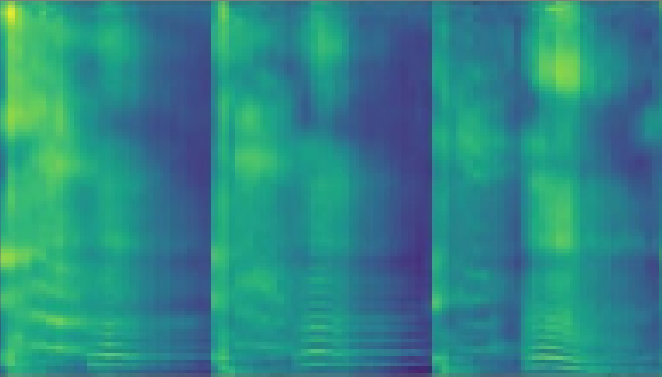E. Haritaoglu, N. Rasmussen, D. Tan, J. Ranjani, J. Xiao, G. Chaudhari, A. Rajput, P. Govindan, C. Canham, W. Chen, M. Yamaura, L. Gomezjurado, A. Khanzada, M. Pilanci
Using Deep Learning with Large Aggregated Datasets for COVID-19 Classification from Cough
preprint, 2022
deep learning signal processing self-supervised learning COVID-19
arXiv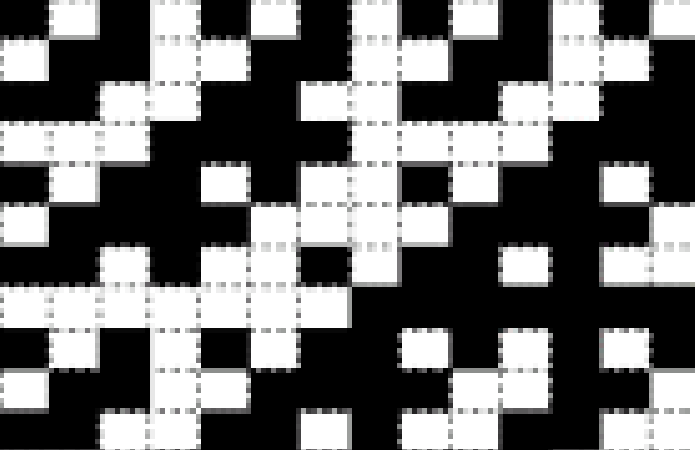N. Charalambides, H. Mahdavifar, M. Pilanci, A. O. Hero III
Orthonormal Sketches for Secure Coded Regression
preprint, 2022
sketching security distributed computing error resilience
arXiv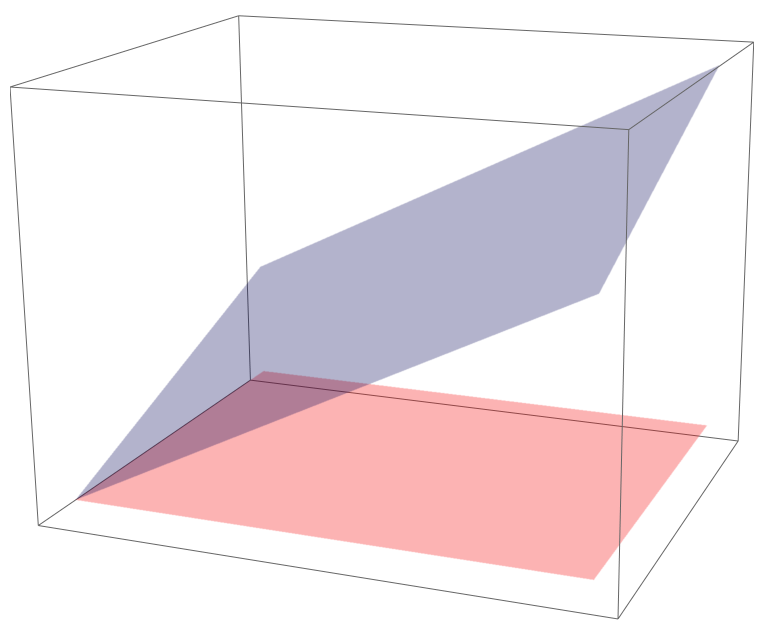J. Lacotte, M. Pilanci
Adaptive and Oblivious Randomized Subspace Methods for High-Dimensional Optimization: Sharp Analysis and Lower Bounds
accepted to IEEE Transactions on Information Theory, 2022
dimension reduction convex optimization randomized SVD kernel methods lower-bounds
PDF arXiv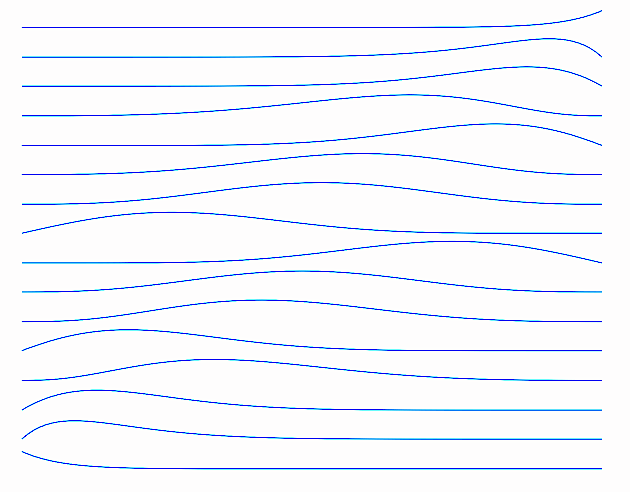M. Pilanci
Computational Polarization: An Information-Theoretic Method for Resilient Computing
IEEE Transactions on Information Theory, 2022
Polar Codes error correcting codes distributed and cloud computing error resilience martingales
PDF arXiv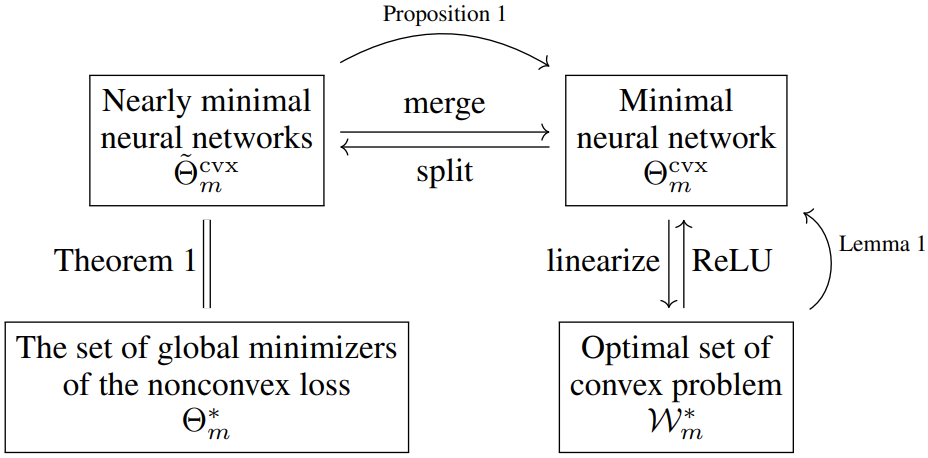Y. Wang, J. Lacotte, M. Pilanci
The Hidden Convex Optimization Landscape of Two-Layer ReLU Neural Networks: an Exact Characterization of the Optimal Solutions
accepted to International Conference on Learning Representations (ICLR), 2022
neural networks convex analysis non-convex non-smooth optimization
arXivA. Sahiner, T. Ergen, B. Ozturkler, B. Bartan, J. Pauly, M. Mardani, M. Pilanci
Hidden Convexity of Wasserstein GANs: Interpretable Generative Models with Closed-Form Solutions
accepted to International Conference on Learning Representations (ICLR), 2022
arXiv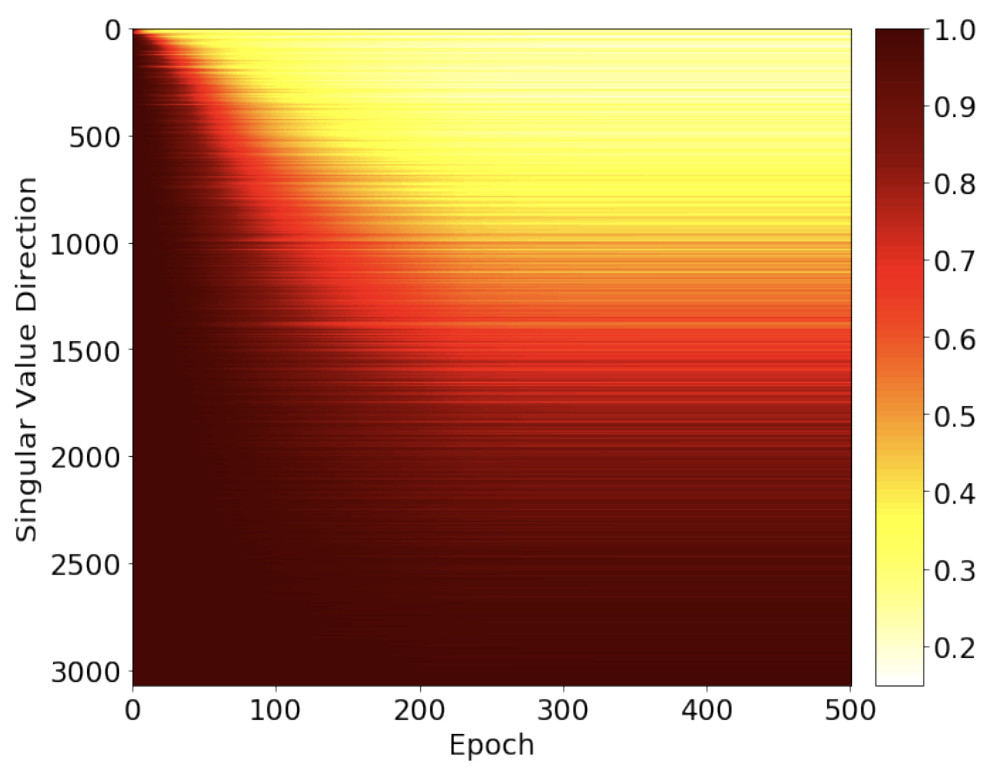T. Ergen, A. Sahiner, B. Ozturkler, J. Pauly, M. Mardani, M. Pilanci
Demystifying Batch Normalization in ReLU Networks: Equivalent Convex Optimization Models and Implicit Regularization
accepted to International Conference on Learning Representations (ICLR), 2022
neural networks deep learning non-convex optimization convexity
arXiv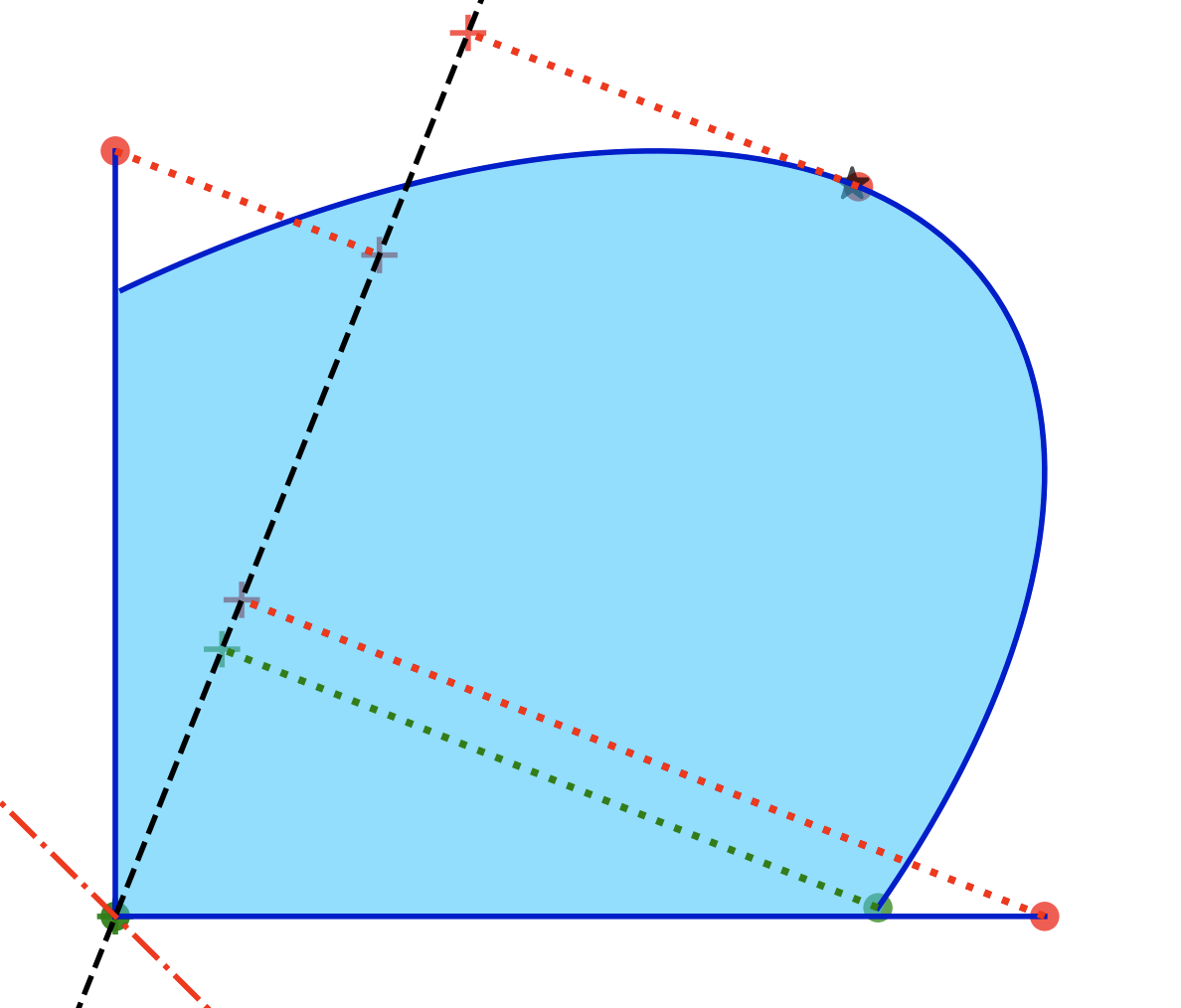Y. Wang, M. Pilanci
The Convex Geometry of Backpropagation: Neural Network Gradient Flows Converge to Extreme Points of the Dual Convex Program
accepted to International Conference on Learning Representations (ICLR), 2022
neural networks non-convex optimization gradient flow convex geometry
arXiv

2021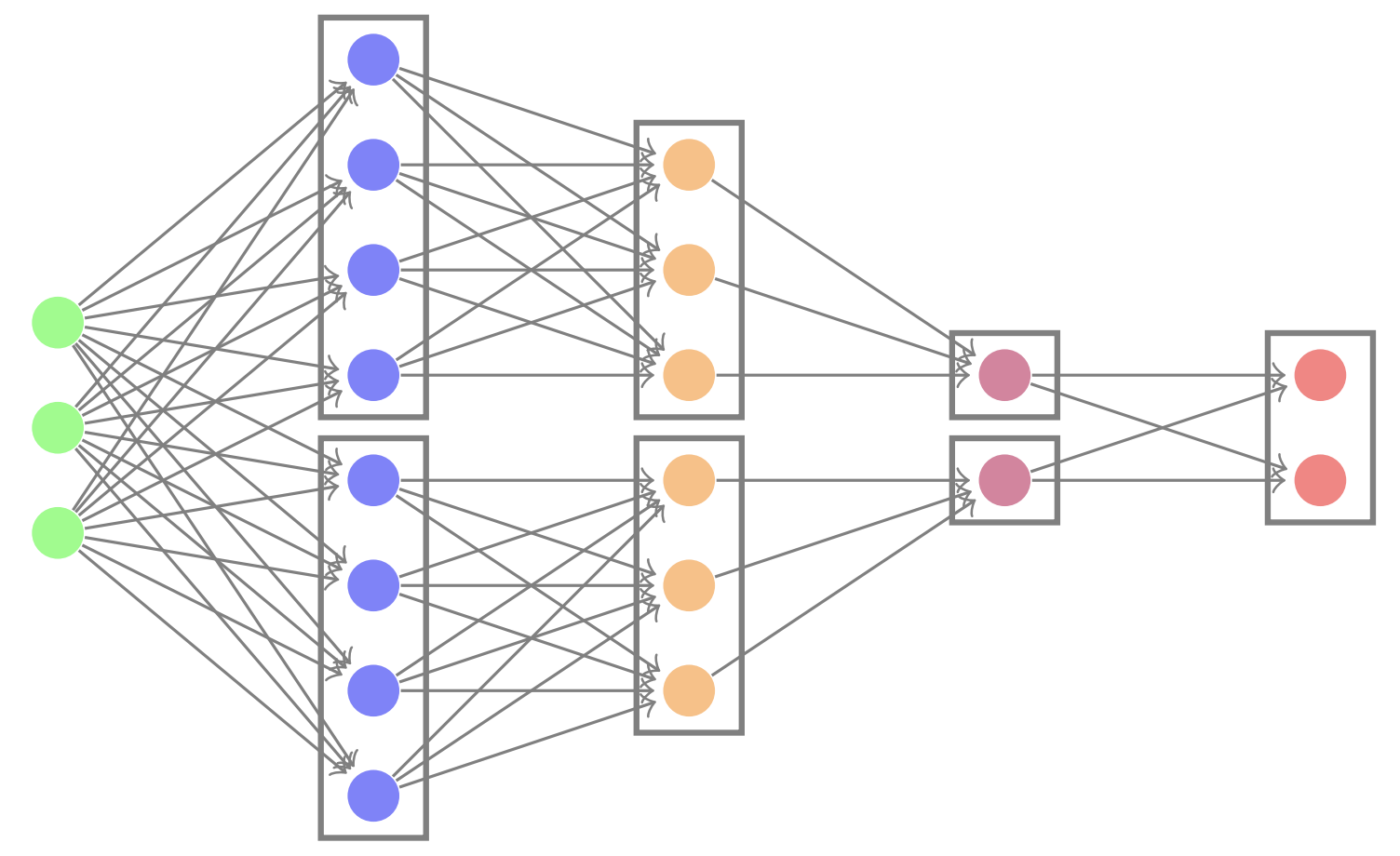Y. Wang, T. Ergen, M. Pilanci
Parallel Deep Neural Networks Have Zero Duality Gap
preprint, 2021
deep neural networks non-convex optimization convex duality
arXiv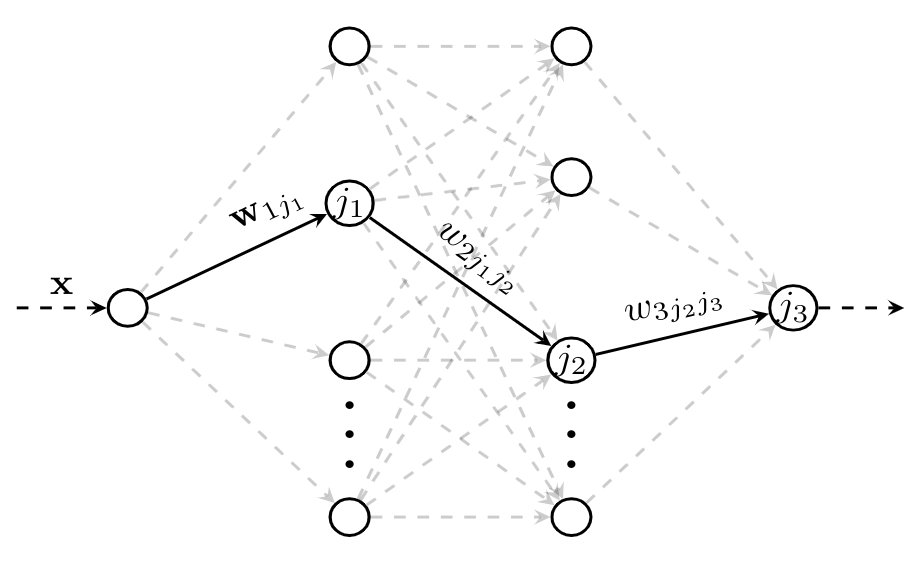T. Ergen, M. Pilanci
Path Regularization: A Convexity and Sparsity Inducing Regularization for Parallel ReLU Networks
preprint, 2021
deep neural networks convex analysis non-convex optimization
arXiv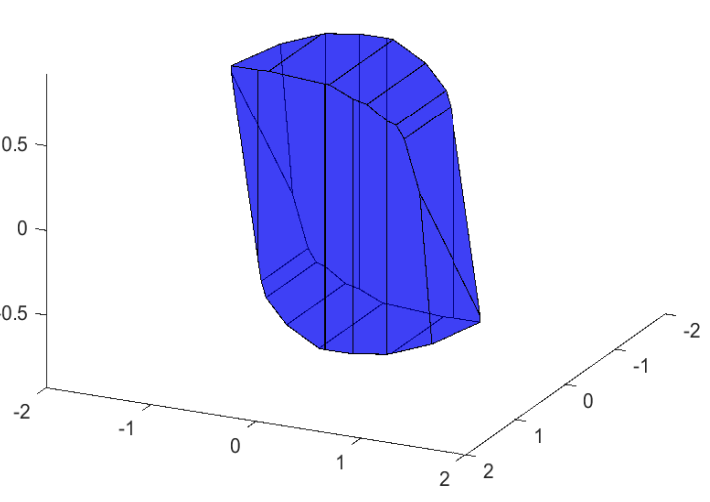T. Ergen, M. Pilanci
Convex Geometry and Duality of Over-parameterized Neural Networks
Journal of Machine Learning Research (JMLR), 2021
neural networks convex analysis non-convex optimization
PDF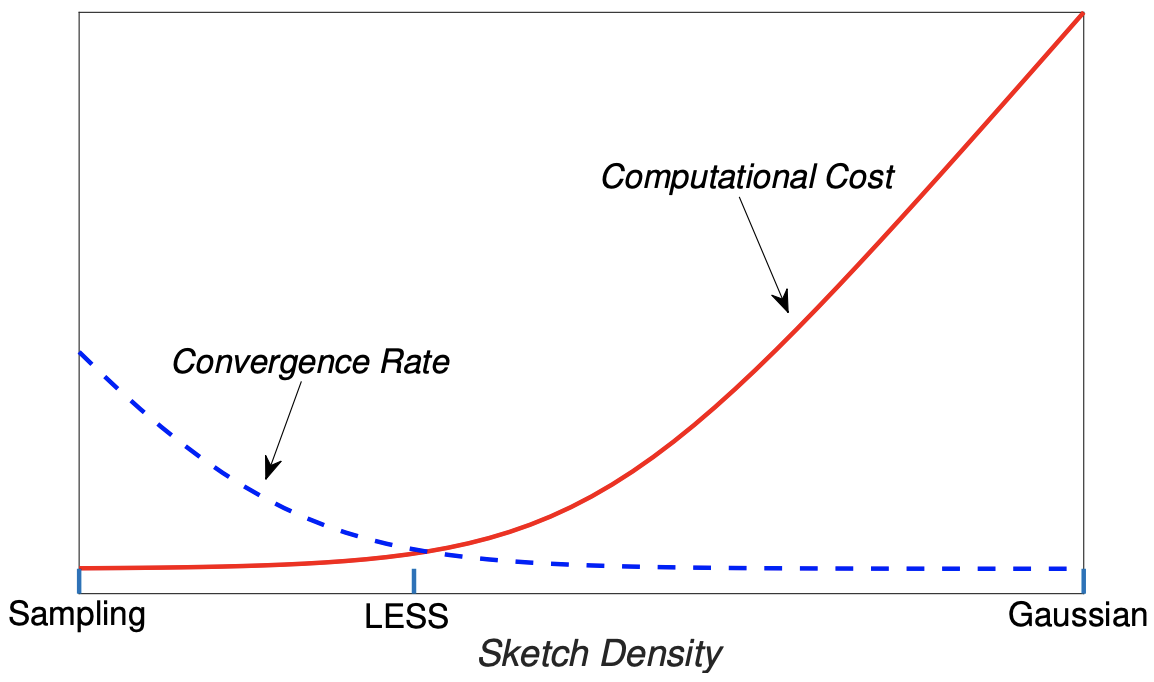M. Dereziński, J. Lacotte, M. Pilanci, M. W. Mahoney
Newton-LESS: Sparsification without Trade-offs for the Sketched Newton Update
Neural Information Processing Systems (NeurIPS), 2021 (spotlight presentation)
randomized algorithms optimization Newton's method
arXiv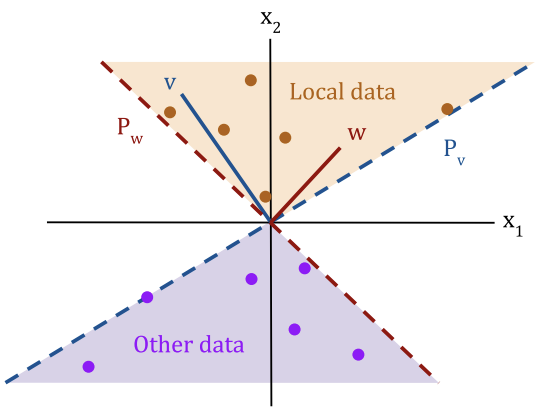V. Gupta, B. Bartan, T. Ergen, M. Pilanci
Convex Neural Autoregressive Models: Towards Tractable, Expressive, and Theoretically-Backed Models for Sequential Forecasting and Generation
IEEE International Conference on Acoustics, Speech and Signal Processing (ICASSP), 2021 (Outstanding Student Paper Award)
generative models neural networks convex optimization
PDF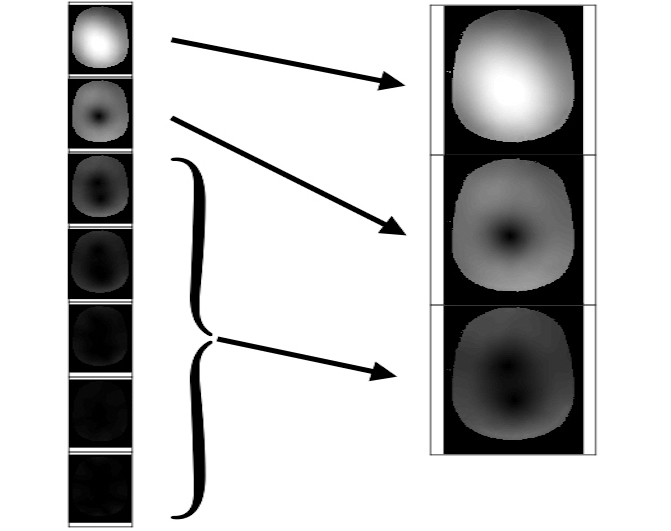J.A. Oscanoa, F. Ong, Z. Li, C.M. Sandino, D.M. Ennis, M. Pilanci, S. Vasanawala
Coil Sketching for fast and memory-efficient iterative reconstruction
In Proceedings of the 29th Annual Meeting of the International Society for Magnetic Resonance in Medicine (ISMRM), 2021
magnetic resonance imaging randomized algorithms sketching inverse problems
PDF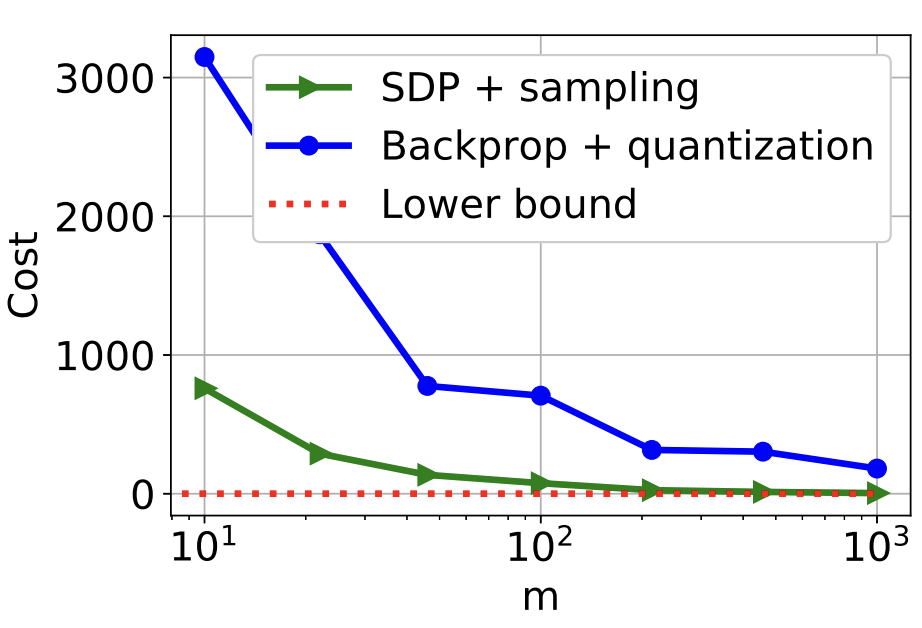B. Bartan, M. Pilanci
Training Quantized Neural Networks to Global Optimality via Semidefinite Programming
International Conference on Machine Learning (ICML), 2021
neural networks quantization semidefinite programming Grothendieck inequality
arXiv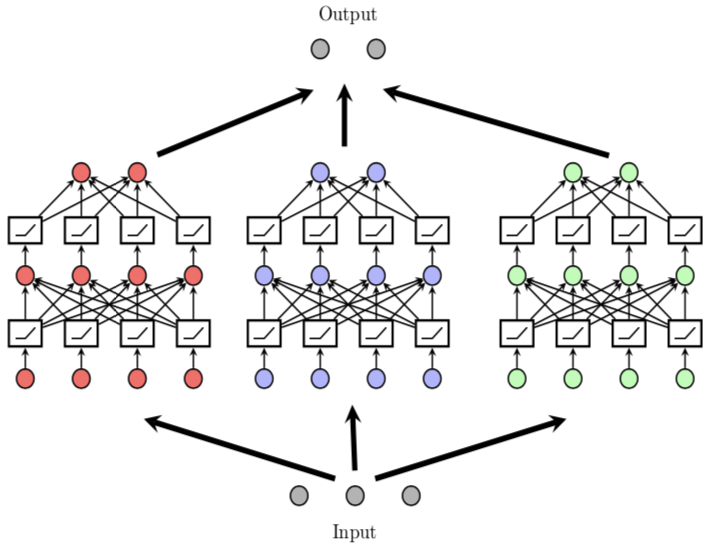T. Ergen, M. Pilanci
Global Optimality Beyond Two Layers: Training Deep ReLU Networks via Convex Programs
International Conference on Machine Learning (ICML), 2021
deep neural networks convex optimization convex regularization
pdfJ. Lacotte, Y. Wang, M. Pilanci
Adaptive Newton Sketch: Linear-time Optimization with Quadratic Convergence and Effective Hessian Dimensionality
International Conference on Machine Learning (ICML), 2021
convex optimization Newton's method randomized algorithms sketching
pdf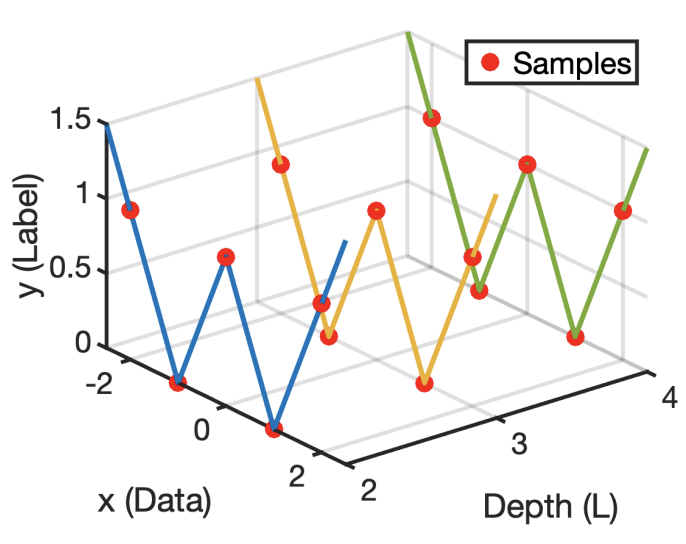T. Ergen, M. Pilanci
Revealing the Structure of Deep Neural Networks via Convex Duality
International Conference on Machine Learning (ICML), 2021
deep neural networks convex analysis non-convex optimization
arXiv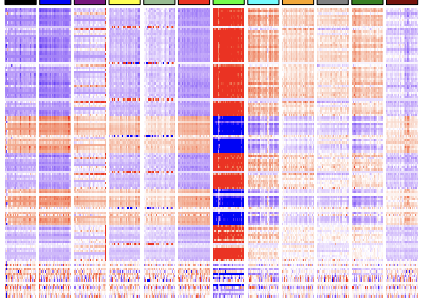L. Liu, X. Zhan, R. Wu, X. Guan, Z. Wang, W. Zhang, M. Pilanci, Y. Wang, Z. Luo, G. Li
Boost AI Power: Data Augmentation Strategies with Unlabelled Data and Conformal Prediction, a Case in Alternative Herbal Medicine Discrimination with Electronic Nose
IEEE Sensors Journal, 2021
deep neural networks convex analysis non-convex optimization
pdf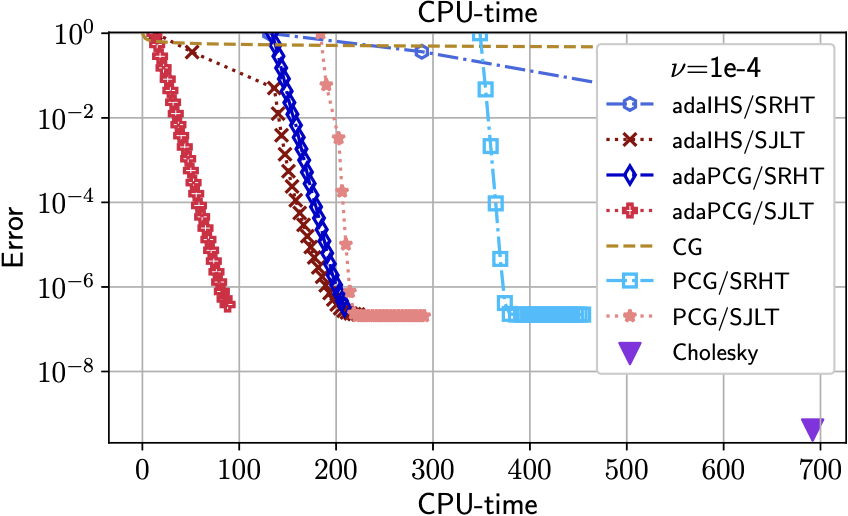J. Lacotte, M. Pilanci
Preprint, 2021
linear solvers optimization preconditioning randomized algorithms
arXiv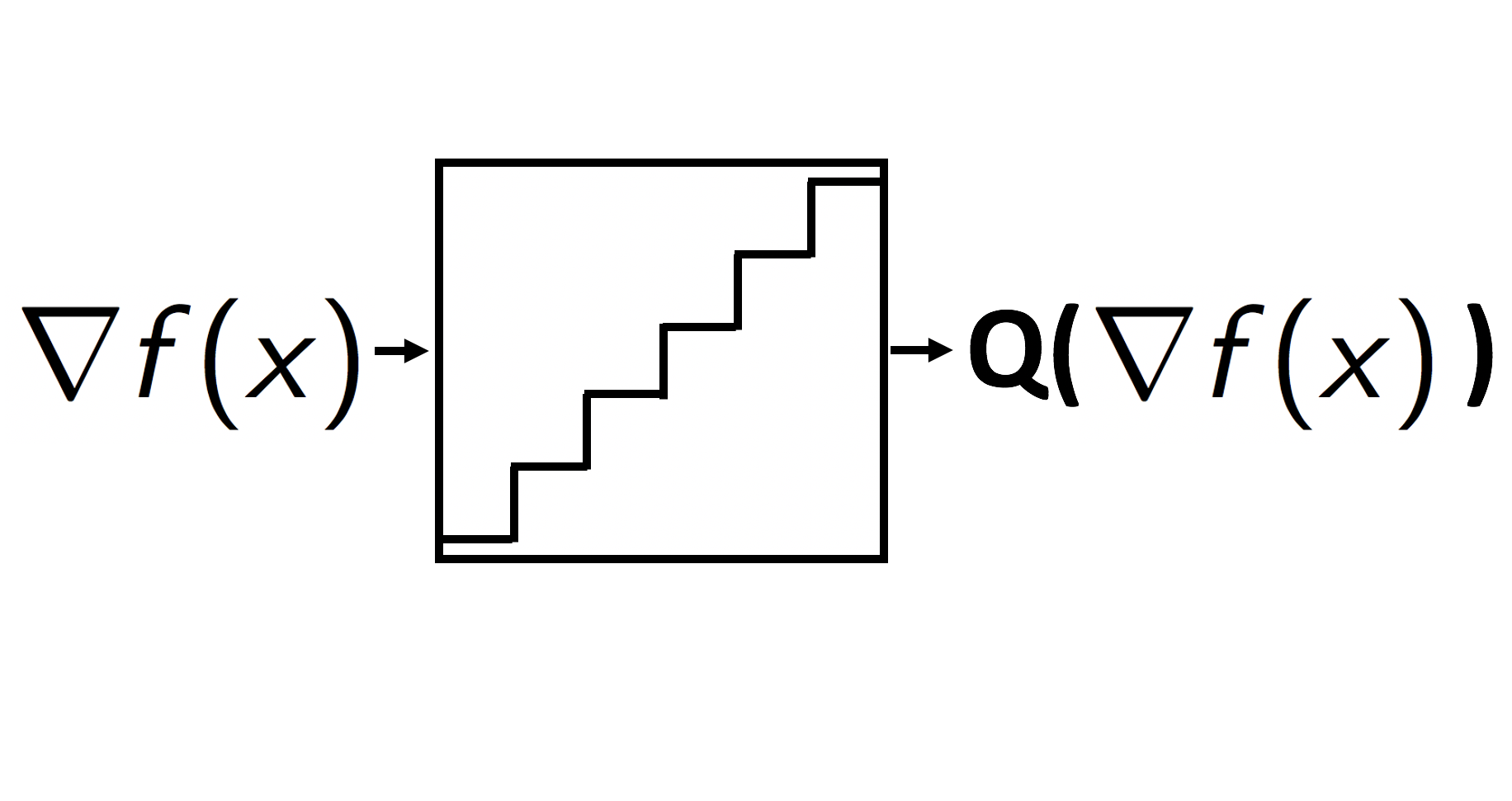R. Saha, M. Pilanci, A. J. Goldsmith
Distributed Learning and Democratic Embeddings: Polynomial-Time Source Coding Schemes Can Achieve Minimax Lower Bounds for Distributed Gradient Descent under Communication Constraints
Preprint, 2021
optimization quantization source coding information theory
arXiv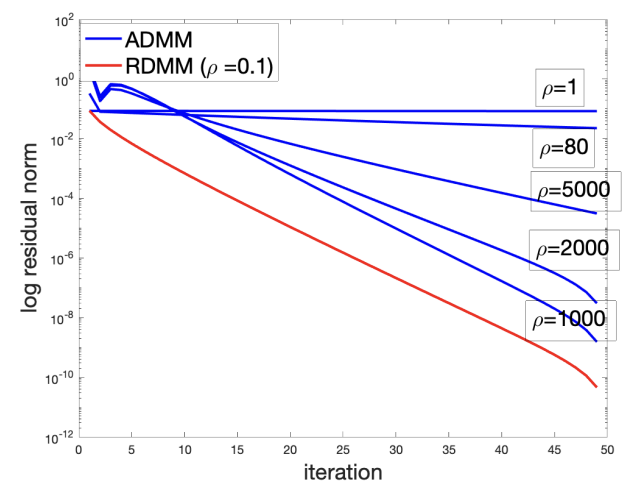E. J. Candès, Q. Jiang, M. Pilanci
Randomized Alternating Direction Methods for Efficient Distributed Optimization
Preprint, 2021
randomized algorithms distributed optimization
PDF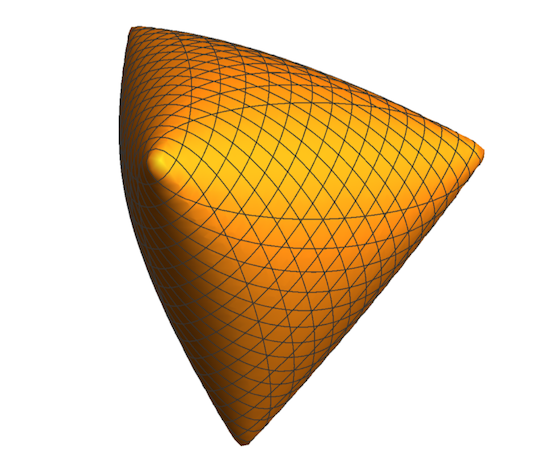B. Bartan, M. Pilanci
Neural Spectrahedra and Semidefinite Lifts: Global Convex Optimization of Polynomial Activation Neural Networks in Fully Polynomial-Time
Preprint, 2021
neural networks non-convex optimization computational complexity semidefinite programming
PDF arXiv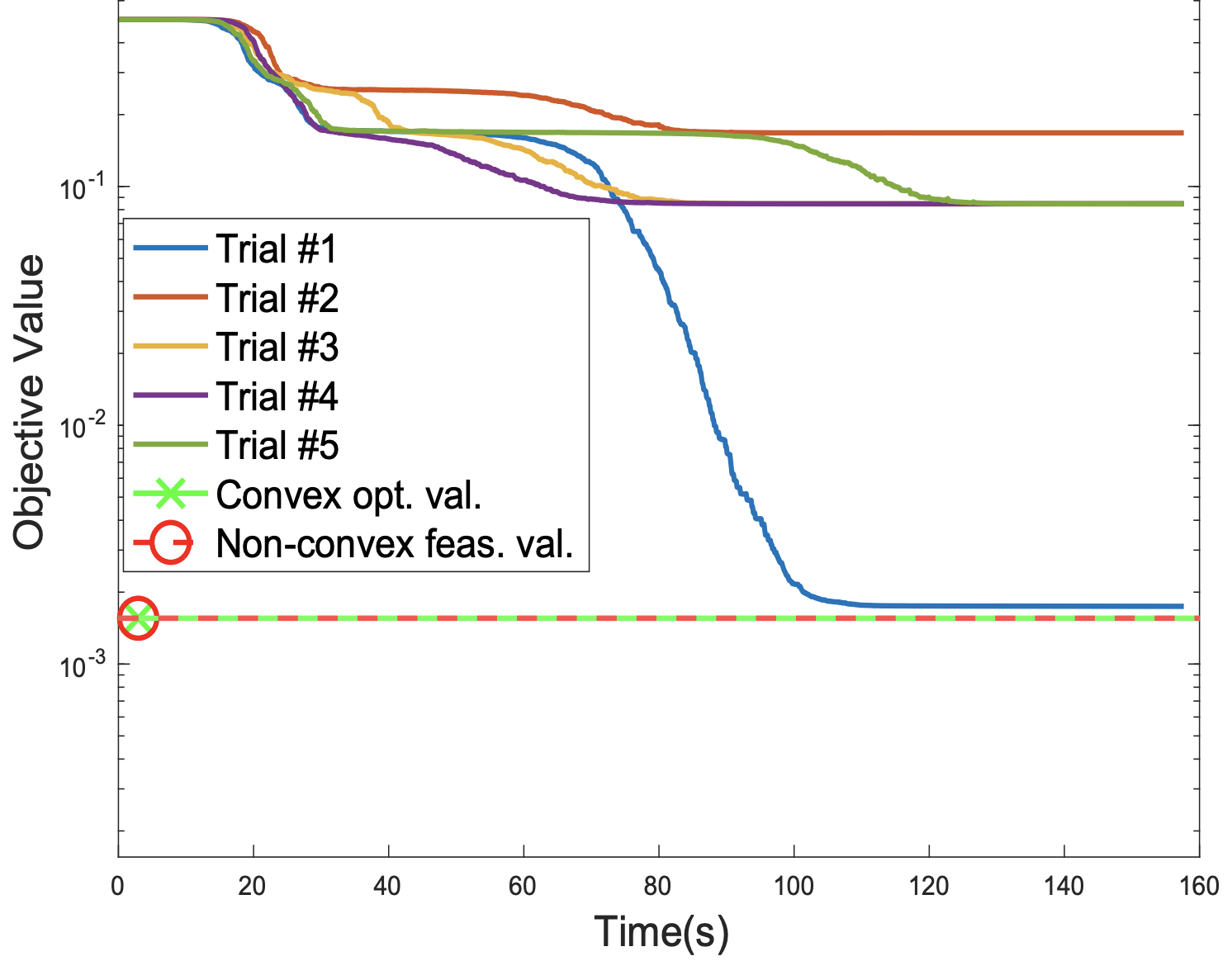T. Ergen, M. Pilanci
Implicit Convex Regularizers of CNN Architectures: Convex Optimization of Two- and Three-Layer Networks in Polynomial Time
International Conference on Learning Representations, ICLR 2021 (spotlight presentation)
convolutional neural networks convex optimization deep learning
arXiv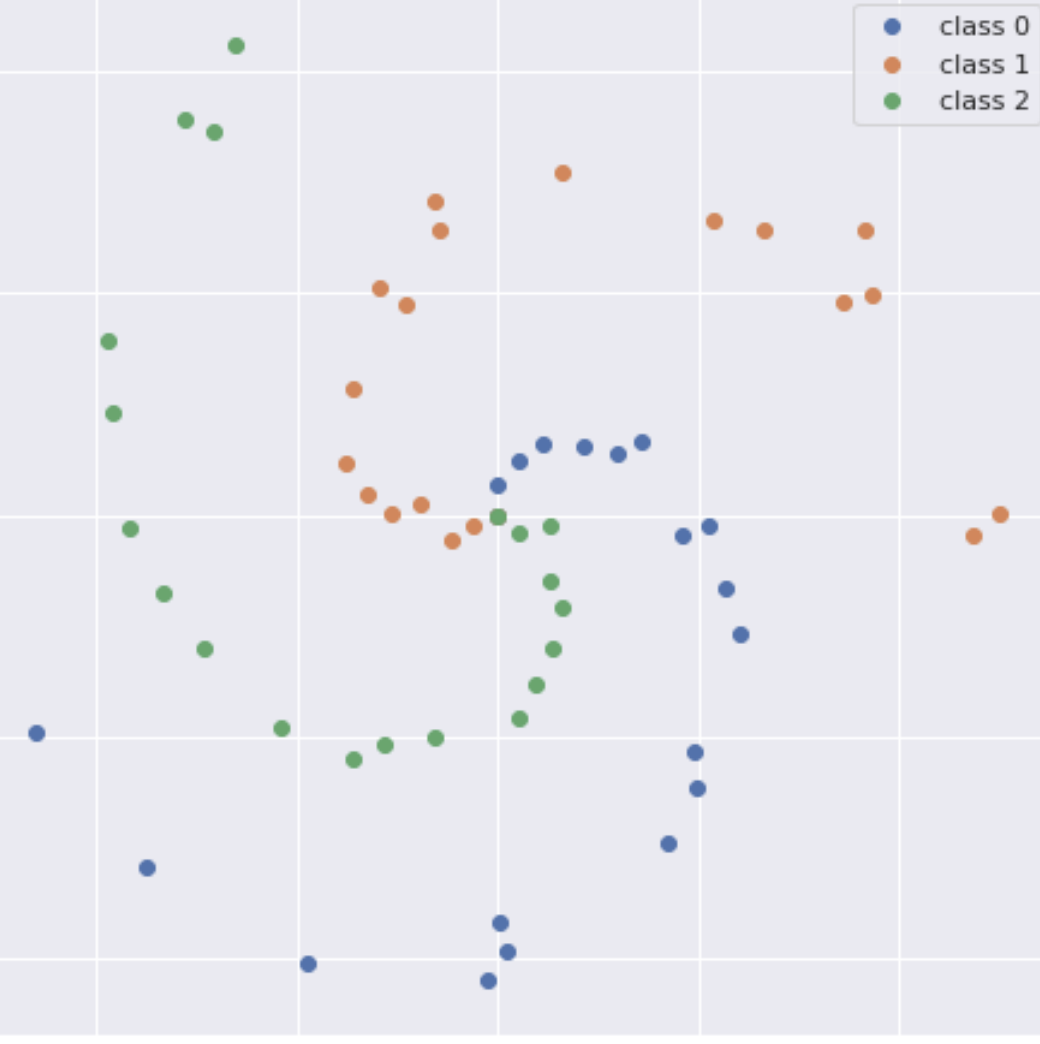A. Sahiner, T. Ergen, J. Pauly, M. Pilanci
Vector-output ReLU Neural Network Problems are Copositive Programs: Convex Analysis of Two Layer Networks and Polynomial-time Algorithms
International Conference on Learning Representations, ICLR 2021
neural networks non-convex optimization copositive programming
arXiv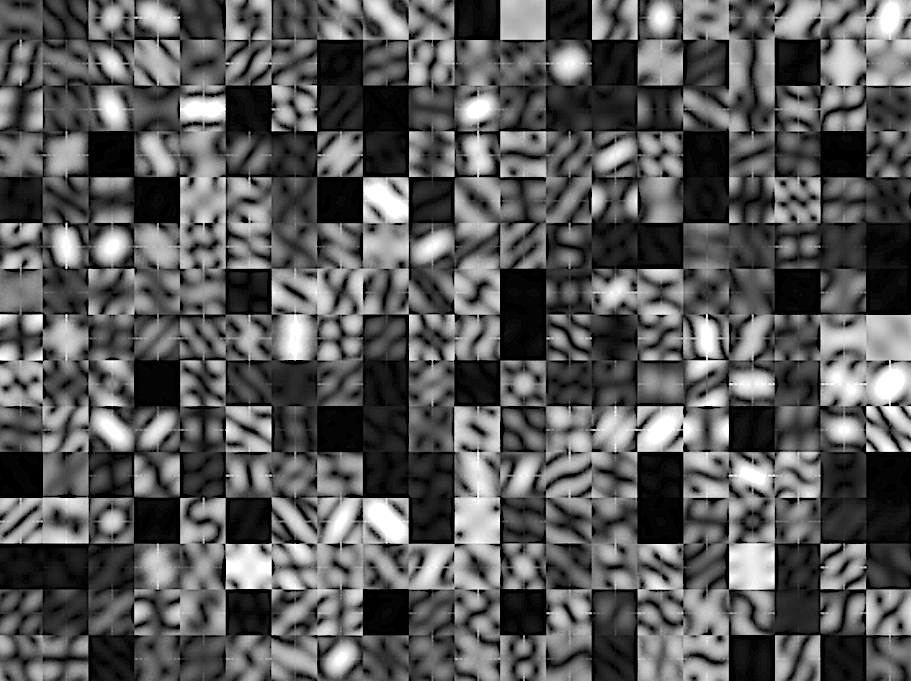A. Sahiner, M. Mardani, B. Ozturkler, M. Pilanci, J. Pauly
Convex Regularization behind Neural Reconstruction
International Conference on Learning Representations, ICLR 2021
neural networks inverse problems convex duality
arXiv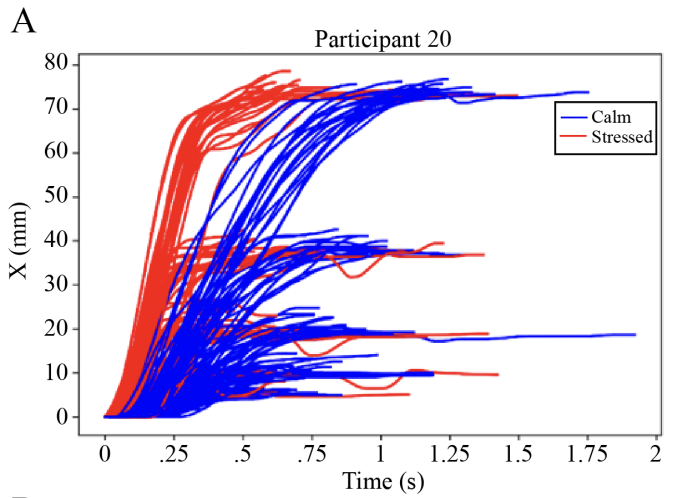L. Kim, R. Goel, J. Liang, M. Pilanci, P. Paredes
Linear Predictive Coding as a Valid Approximation of a Mass Spring Damper Model for Acute Stress Prediction from Computer Mouse Movement
43rd Annual International Conference of the IEEE Engineering in Medicine and Biology Society, 2021
signal processing passive sensing neural networks
arXiv

2020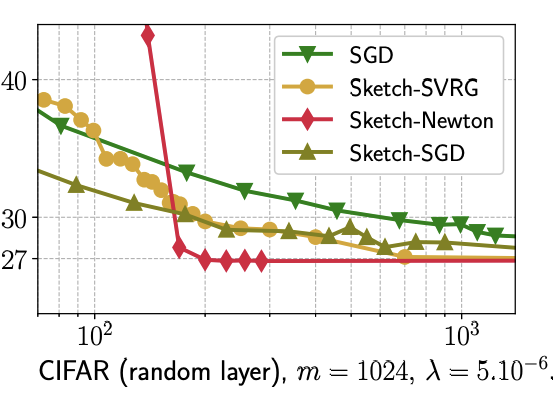J. Lacotte, M. Pilanci
Adaptive and Oblivious Randomized Subspace Methods for High-Dimensional Optimization: Sharp Analysis and Lower Bounds
Preprint, 2020
randomized algorithms high dimensional optimization lower-bounds
arXiv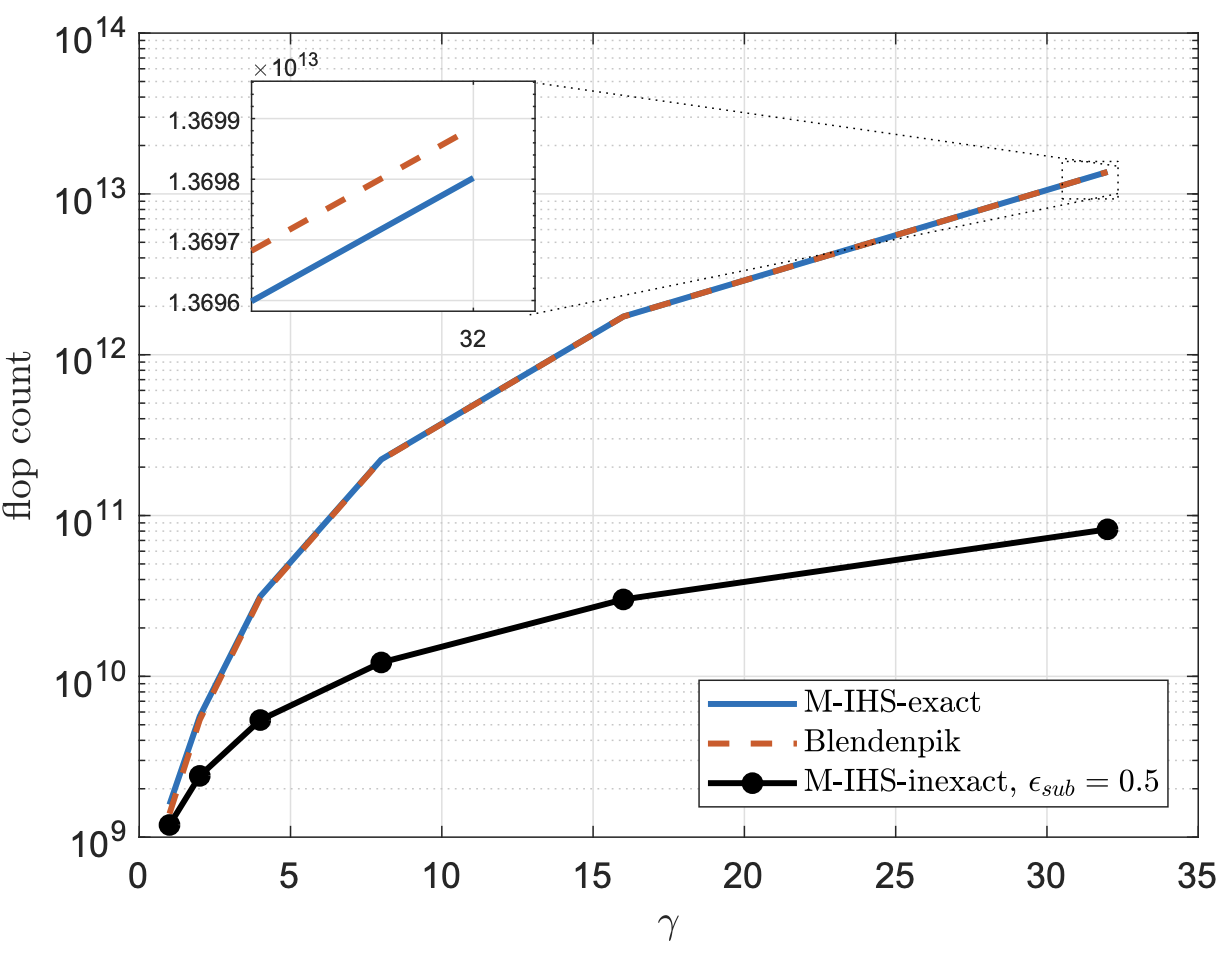I.K. Ozaslan, M. Pilanci, O. Arikan
M-IHS: An Accelerated Randomized Preconditioning Method Avoiding Costly Matrix Decompositions
Preprint, 2020
randomized algorithms iterative linear solvers preconditioning
arXiv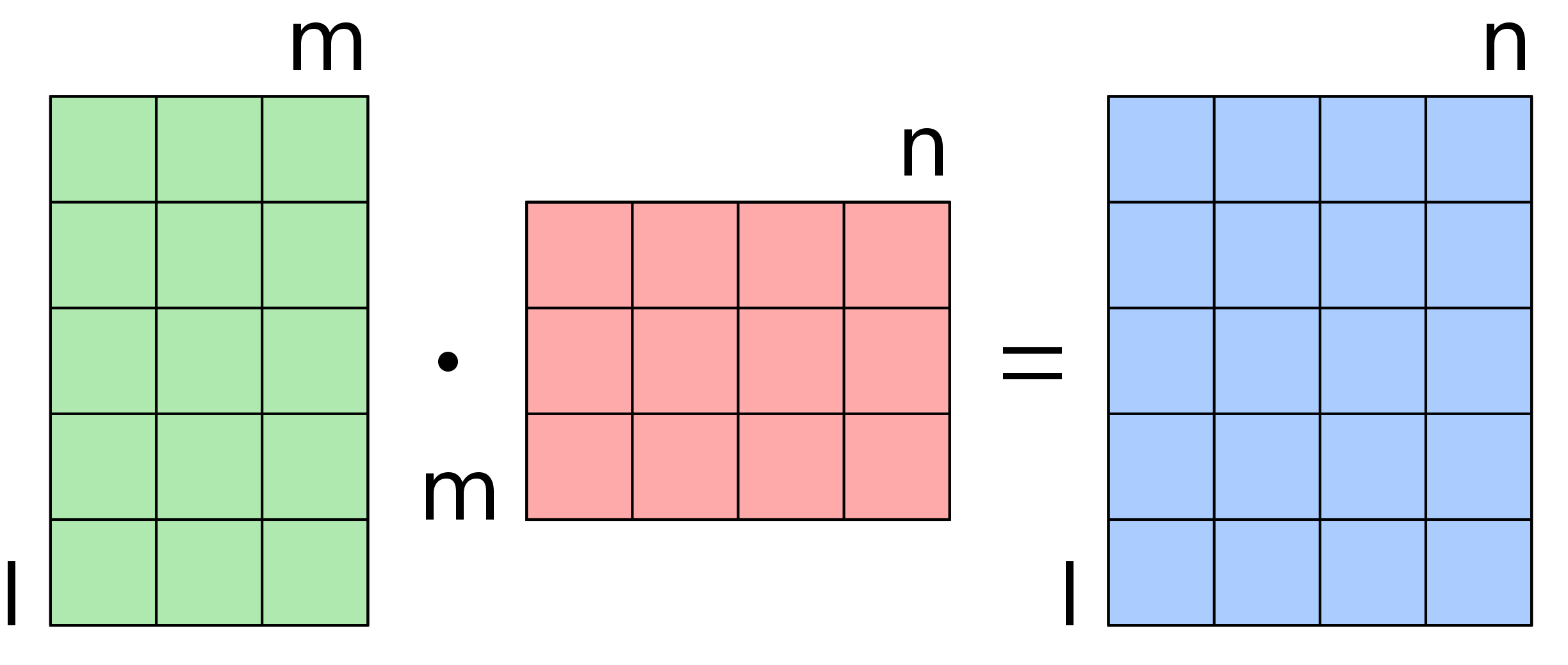N. Charalambides, M. Pilanci, A. O. Hero III
Approximate Weighted CR Coded Matrix Multiplication
IEEE International Conference on Acoustics, Speech and Signal Processing (ICASSP), 2021
distributed computing randomized linear algebra
arXiv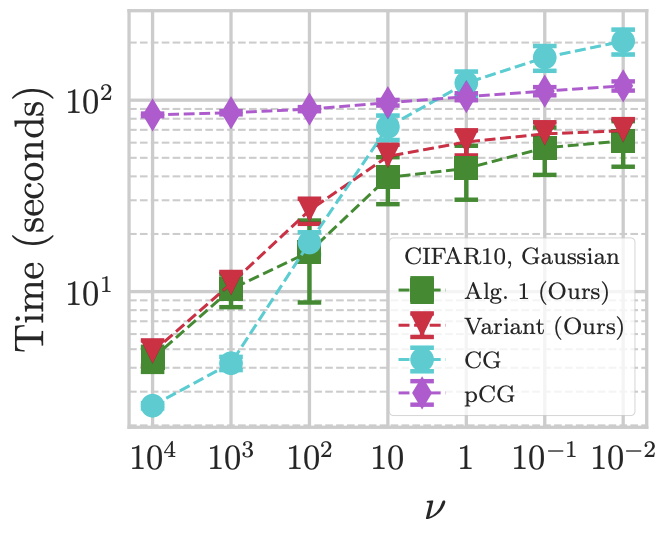J. Lacotte, M. Pilanci
Effective Dimension Adaptive Sketching Methods for Faster Regularized Least-Squares Optimization
NeurIPS 2020 (oral presentation)
randomized algorithms random projection random matrix theory
arXiv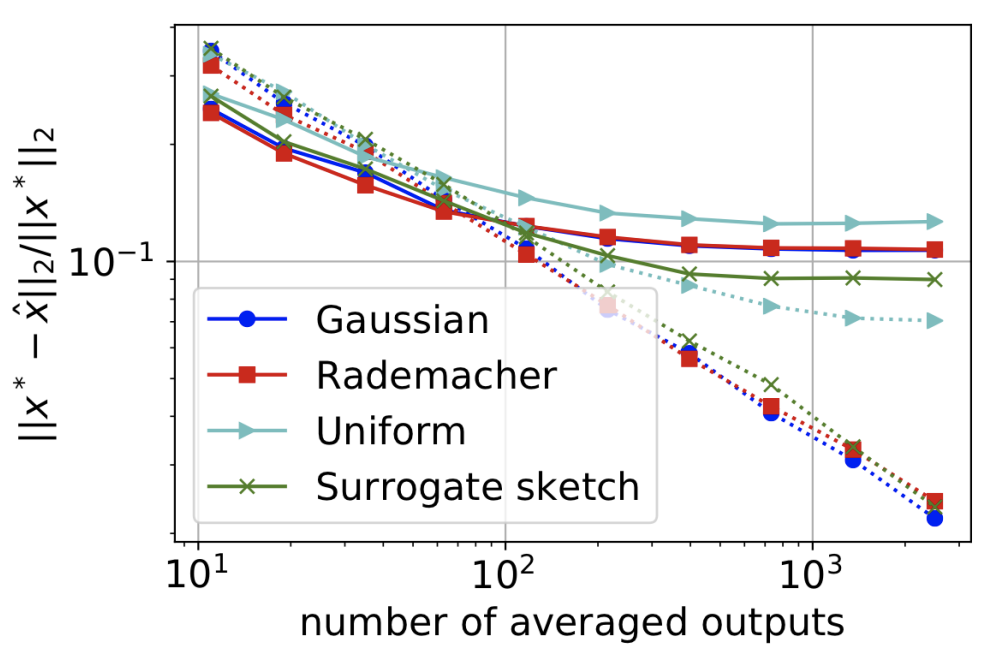M. Derezinski, B. Bartan, M. Pilanci, M. Mahoney
Debiasing Distributed Second Order Optimization with Surrogate Sketching and Scaled Regularization
NeurIPS 2020
randomized algorithms distributed optimization determinantal point processes
arXiv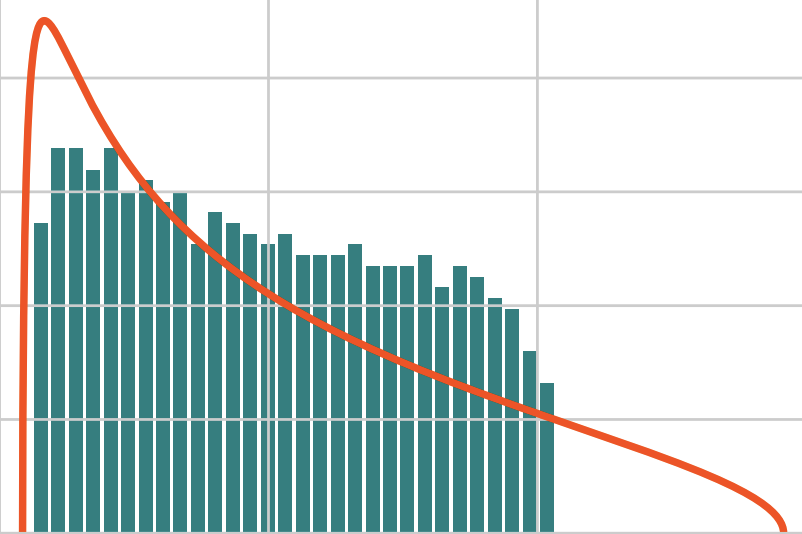J. Lacotte, S. Liu, E. Dobriban, M. Pilanci
Limiting Spectrum of Randomized Hadamard Transform and Optimal Iterative Sketching Methods
NeurIPS 2020
random matrix theory free probability randomized algorithms
arXivS. Sridhar, M. Pilanci, A. Ozgur
Lower Bounds and a Near-Optimal Shrinkage Estimator for Least Squares using Random Projections
IEEE Journal on Selected Areas in Information Theory 2020
randomized algorithms Stein's paradox Fisher Information
arXiv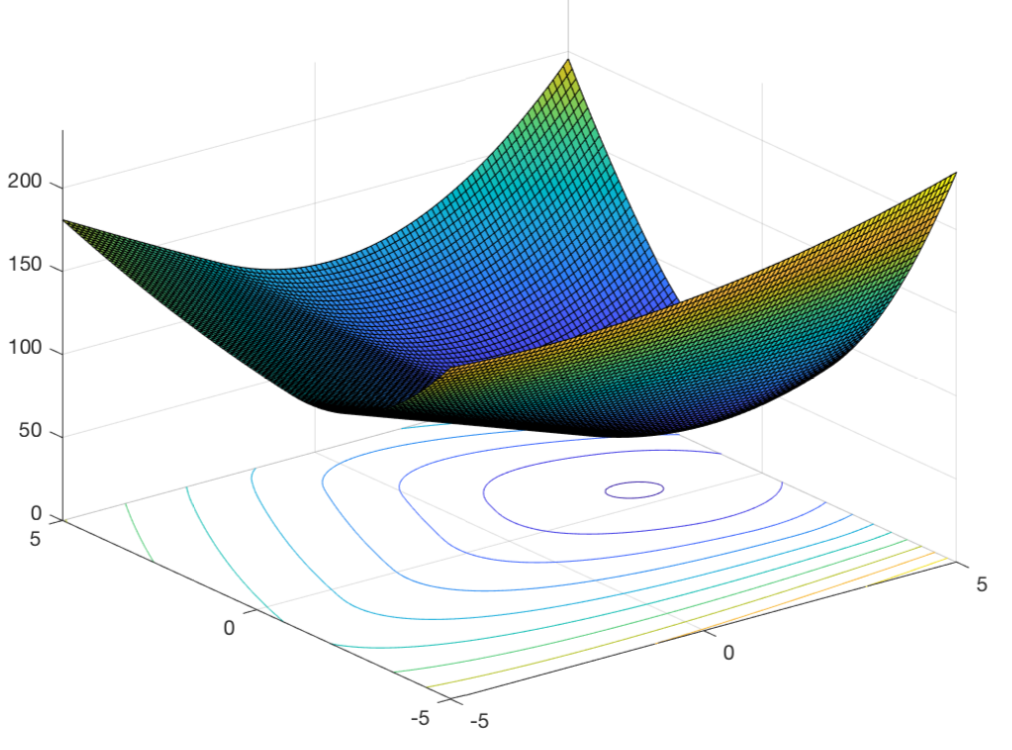J. Lacotte, M. Pilanci
All Local Minima are Global for Two-Layer ReLU Neural Networks: The Hidden Convex Optimization Landscape
preprint, 2020
neural networks convex analysis non-convex non-smooth optimization
arXiv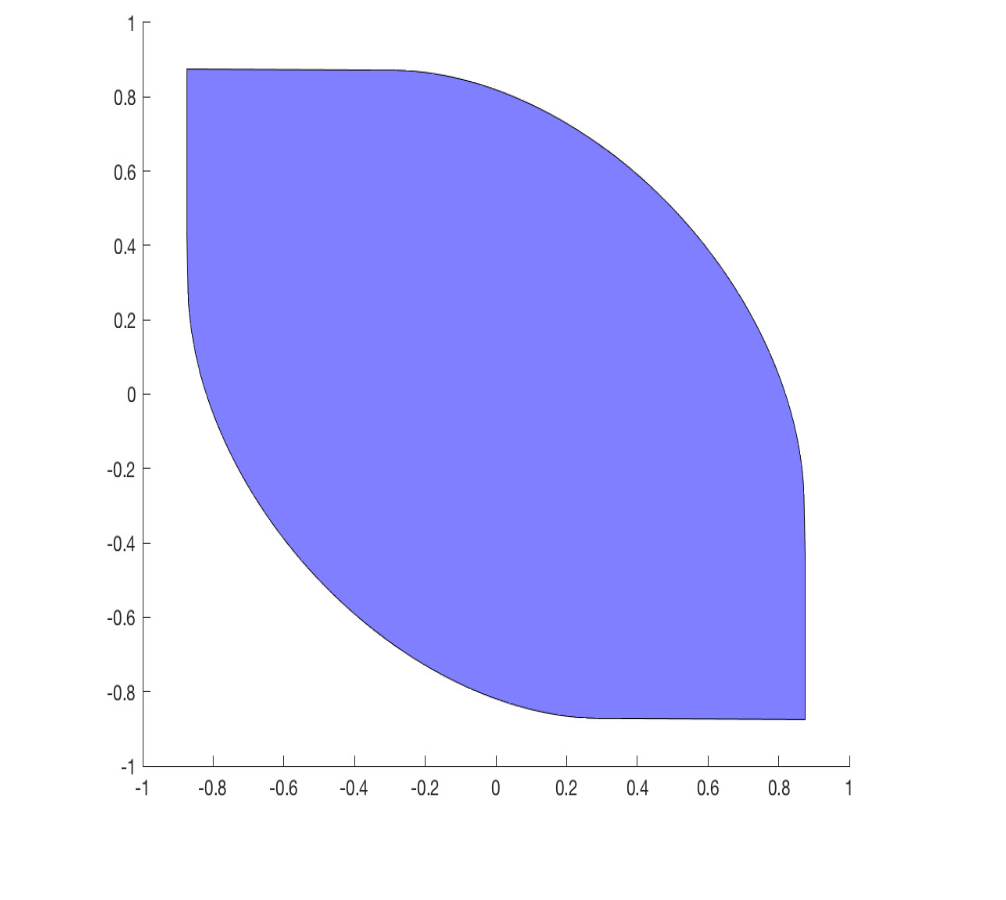M. Pilanci, T. Ergen
Neural Networks are Convex Regularizers: Exact Polynomial-time Convex Optimization Formulations for Two-Layer Networks
International Conference on Machine Learning (ICML), 2020
neural networks convex analysis non-convex optimization
PDF arXiv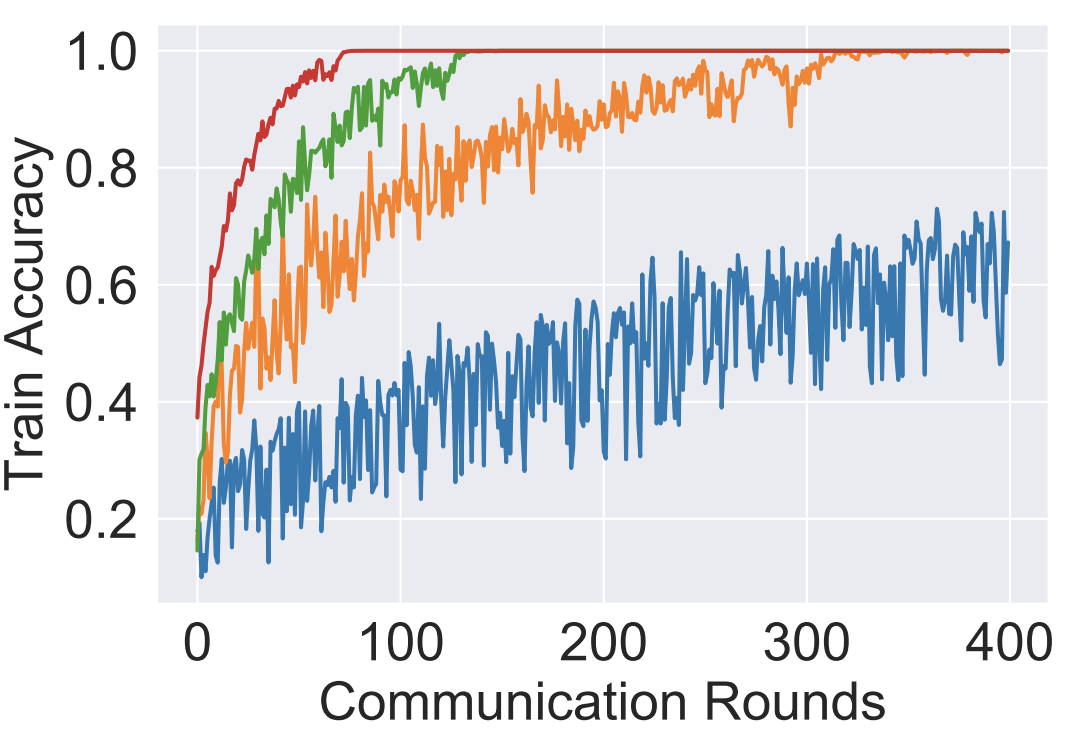S. Ahn, A. Ozgur, M. Pilanci
Global Multiclass Classification from Heterogeneous Local Models
IEEE Journal on Selected Areas in Information Theory 2020
information theory federated learning
arXiv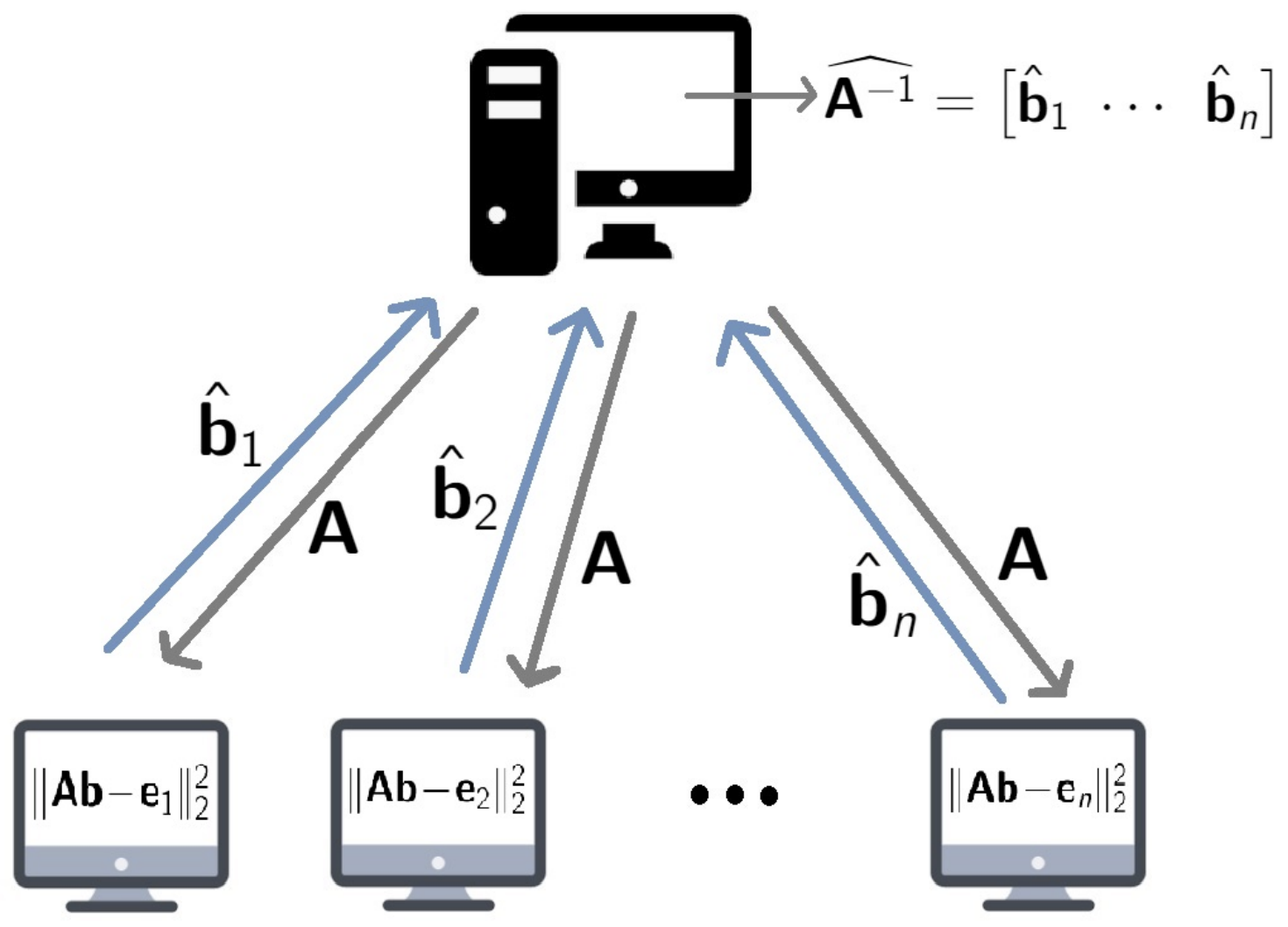N. Charalambides, M. Pilanci, A. O. Hero III
Straggler Robust Distributed Matrix Inverse Approximation
Preprint, 2020
distributed computing error resilience
arXiv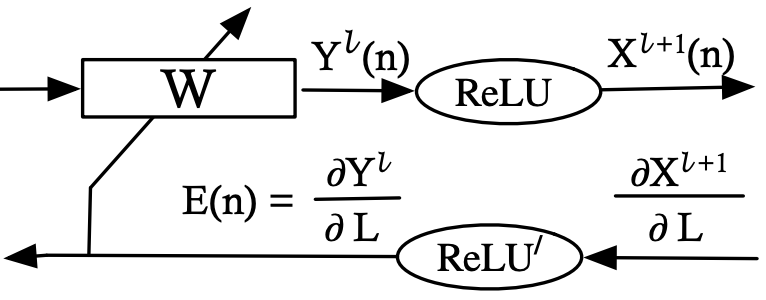E. Chai, M. Pilanci , B. Murmann
Separating the Effects of Batch Normalization on CNN Training Speed and Stability Using Classical Adaptive Filter Theory
Asilomar 2020
arXiv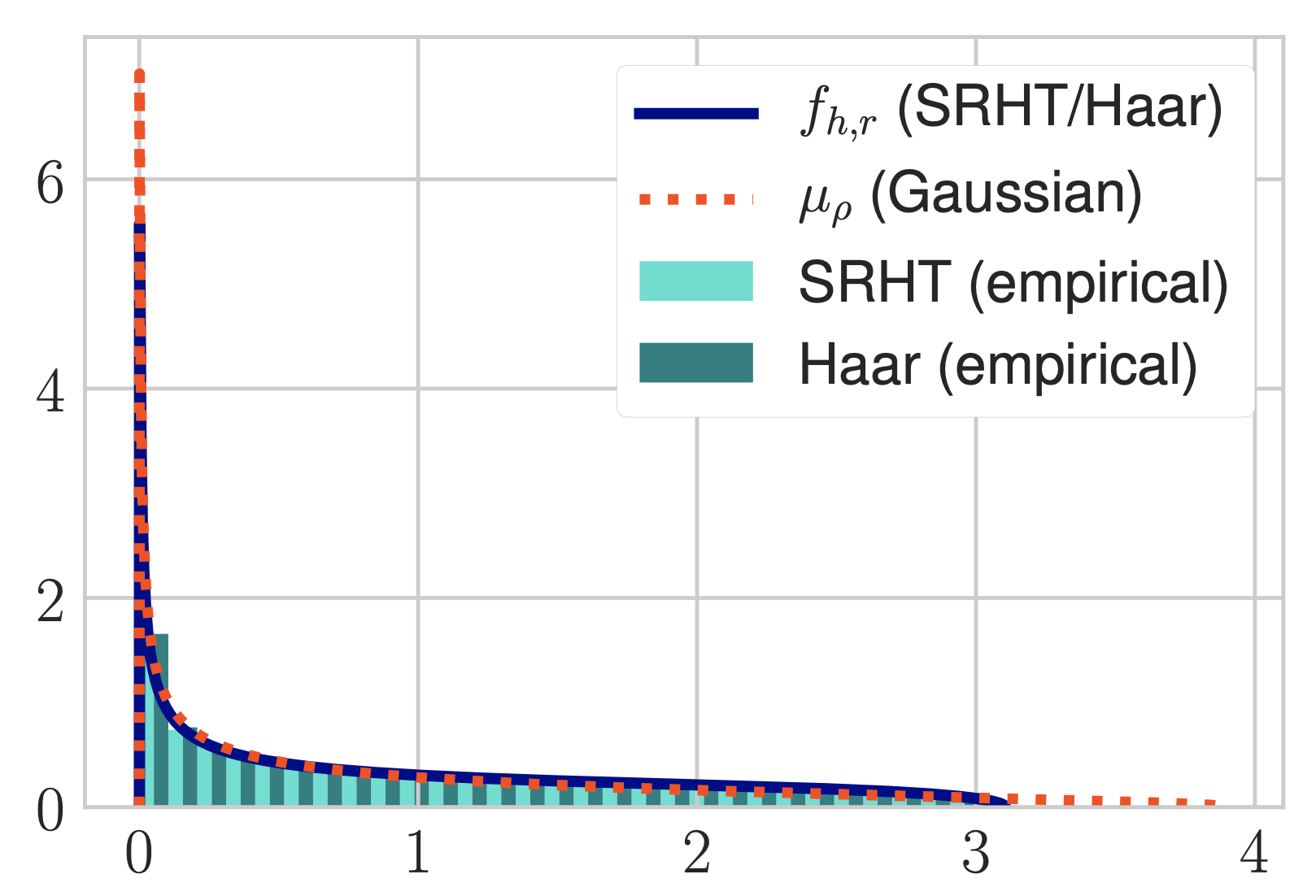J. Lacotte, M. Pilanci
Optimal Randomized First-Order Methods for Least-Squares Problems
International Conference on Machine Learning (ICML), 2020
convex optimization randomized algorithms orthogonal polynomials
arXiv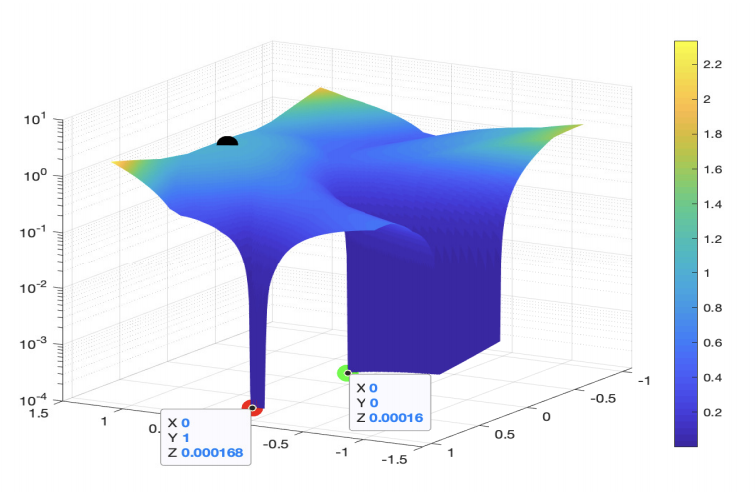T. Ergen, M. Pilanci
Convex geometry of two-layer relu networks: Implicit autoencoding and interpretable models
23rd International Conference on Artificial Intelligence and Statistics (AISTATS 2020)
neural networks convex analysis non-convex optimization
PDF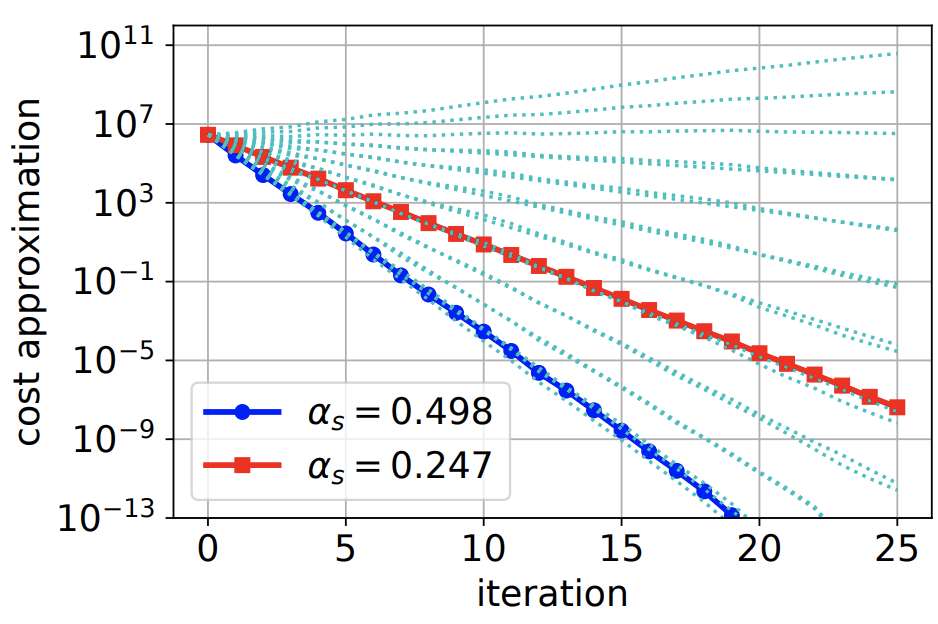B. Bartan, M. Pilanci
Distributed Averaging Methods for Randomized Second Order Optimization
Preprint, 2020
distributed optimization randomized algorithms serverless computing
arXiv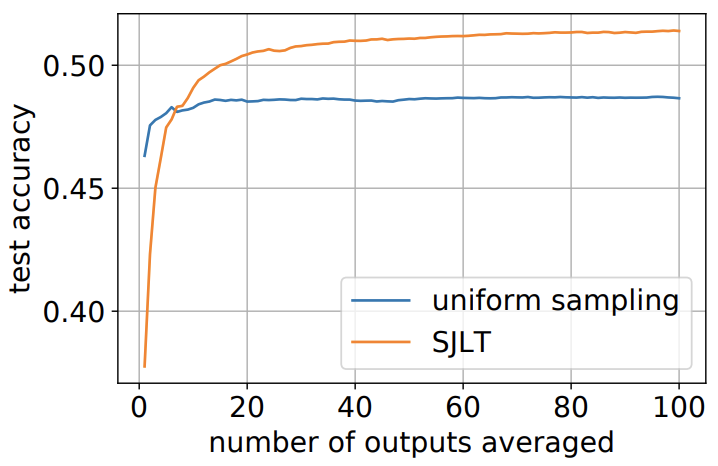B. Bartan, M. Pilanci
Distributed Sketching Methods for Privacy Preserving Regression
Preprint, 2020
machine learning randomized algorithms serverless computing
arXiv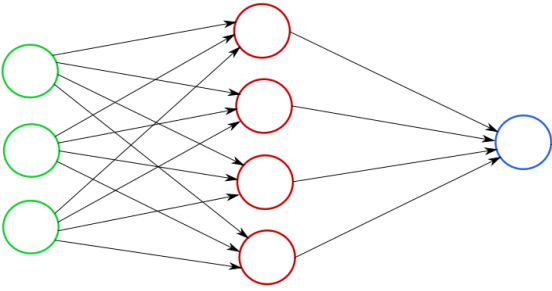A. d'Aspremont, M. Pilanci
Global Convergence of Frank Wolfe on One Hidden Layer Networks
Preprint, 2020
neural networks non-convex optimization
arXiv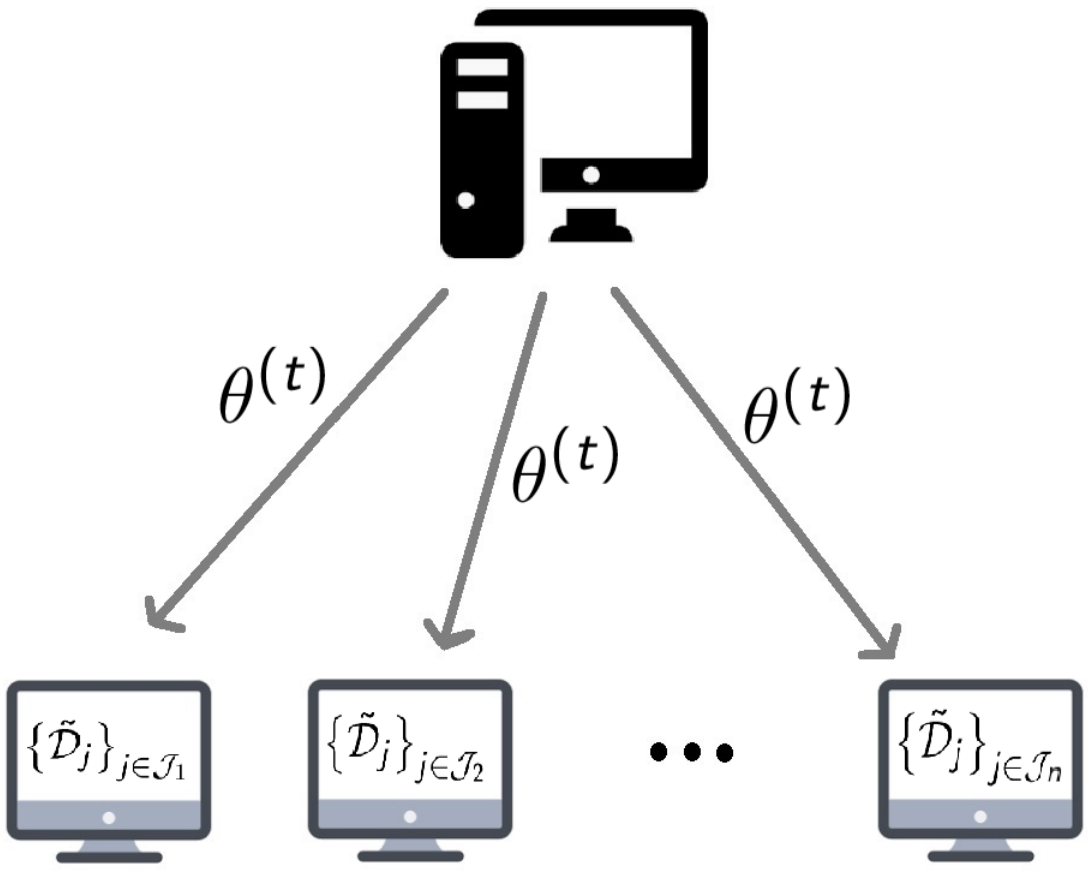N. Charalambides, M. Pilanci, A.O. Hero III
Weighted Gradient Coding with Leverage Score Sampling
Preprint, 2020
coding theory distributed optimization
arXiv

2019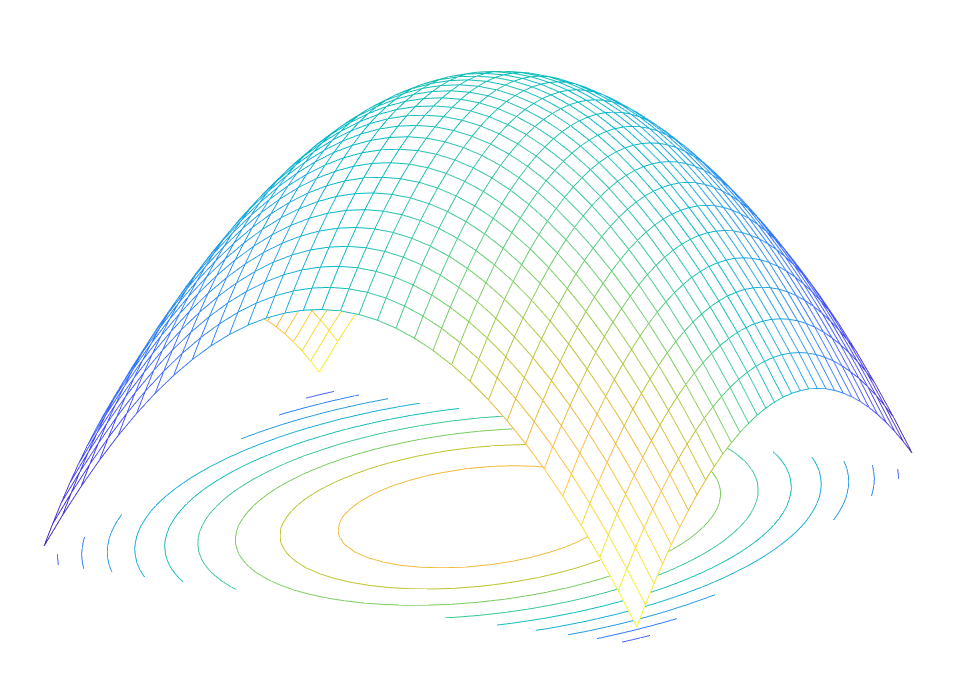J. Lacotte, M. Pilanci
Faster Least Squares Optimization
Preprint, 2019
optimization machine learning computational complexity randomized algorithms
arXiv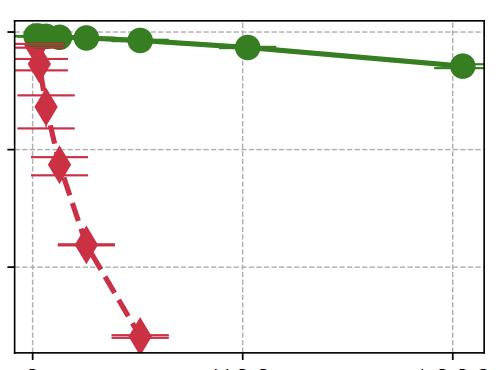J. Lacotte, M. Pilanci, M. Pavone
High-Dimensional Optimization in Adaptive Random Subspaces
Advances in Neural Information Processing Systems (NeurIPS), 2019
optimization machine learning kernel methods randomized algorithms
arXivB. Bartan, M. Pilanci
Distributed Black-Box Optimization via Error Correcting Codes
57th Annual Allerton Conference on Communication, Control, and Computing, 2019
optimization error correcting codes deep learning adversarial examples cloud computing
arXiv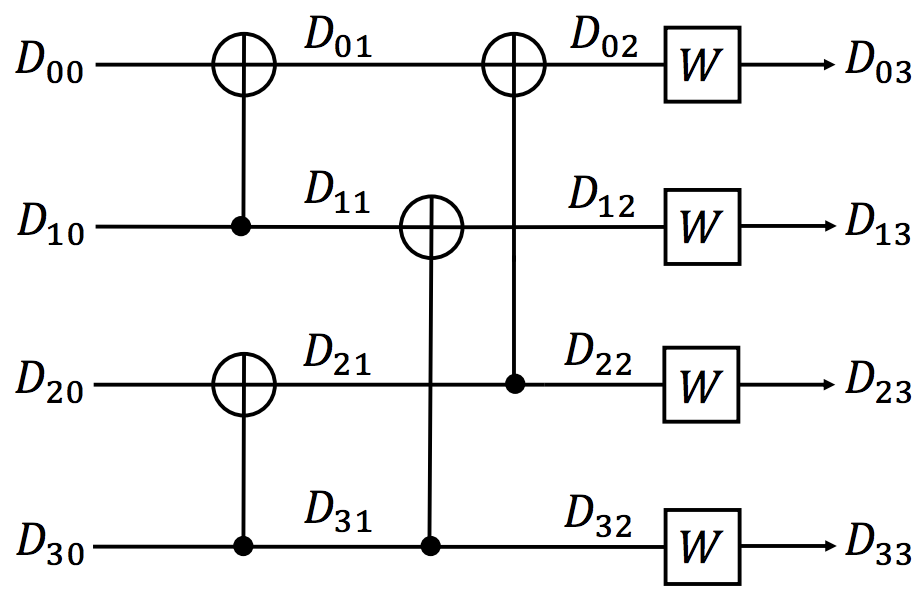B. Bartan and M. Pilanci
Straggler Resilient Serverless Computing Based on Polar Codes
57th Annual Allerton Conference on Communication, Control, and Computing, 2019
polar codes information theory distributed optimization cloud computing
arXiv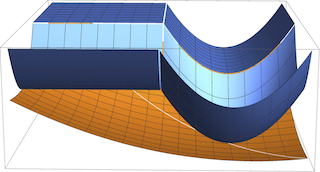B. Bartan and M. Pilanci
Convex Relaxations of Convolutional Neural Nets
IEEE International Conference on Acoustics, Speech and Signal Processing (ICASSP), 2019
neural nets convex optimization
arXiv

2018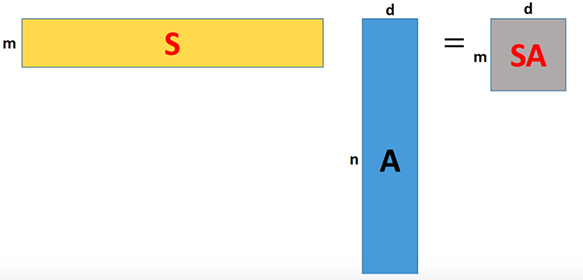M. Pilanci
Information-Theoretic Bounds on Sketching
Book chapter, Information-Theoretic Methods in Data Science, Cambridge University Press, 2021
random projection information theory
PDF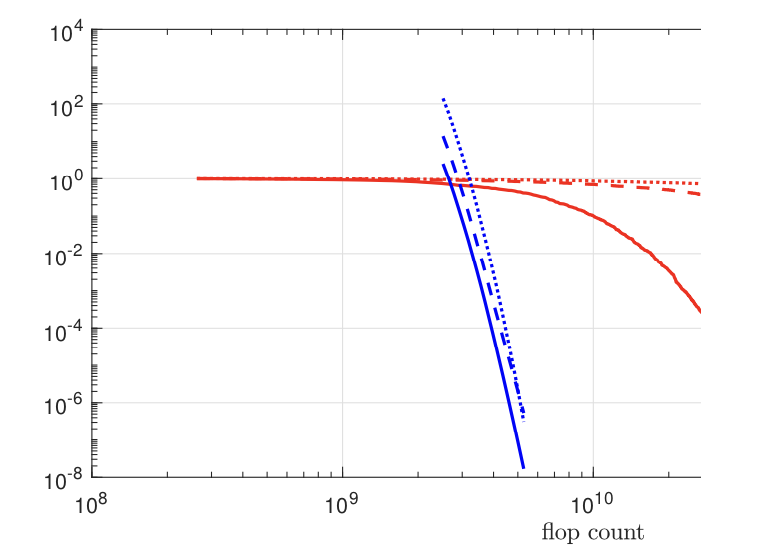I.K. Ozaslan, M. Pilanci and O. Arikan
Iterative Hessian Sketch with Momentum
Preprint, 2018
random projection momentum least squares
PDF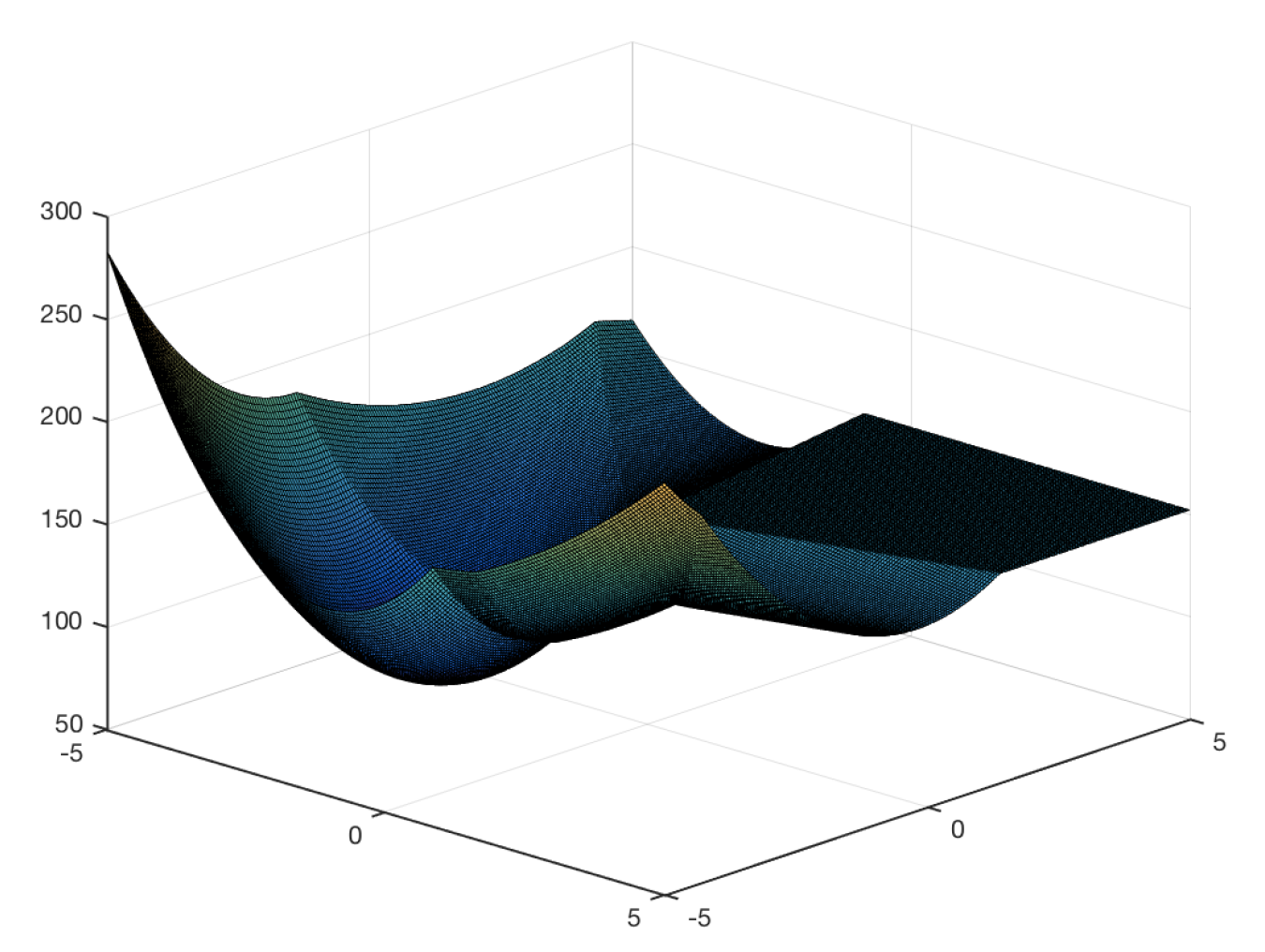M. Pilanci and E. J. Candès
Randomized Methods for Fitting Non-Convex Models
Preprint, 2018
non-convex optimization neural nets phase retrieval

2017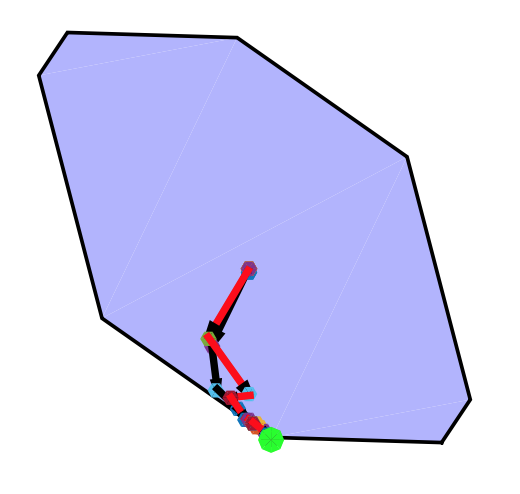M. Pilanci and M. J. Wainwright
Newton Sketch: A Linear-time Optimization Algorithm with Linear-Quadratic Convergence
SIAM Journal of Optimization, SIAM J. Optim., 27(1), 205–245, 2017
randomized algorithms newton's method interior point method convex optimization linear program logistic regression
DOI PDF arXiv

2016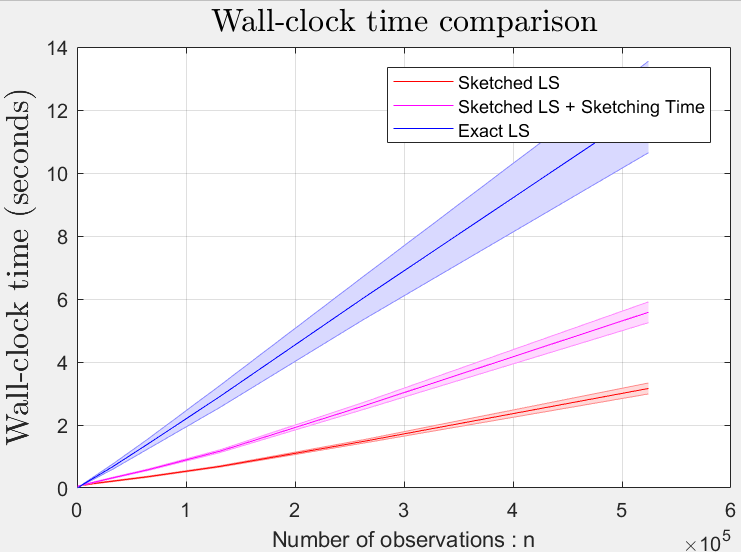M. Pilanci and M. J. Wainwright
Iterative Hessian sketch: Fast and accurate solution approximation for constrained least-squares
Journal of Machine Learning Research (JMLR) 17, 2016
information-theoretic lower-bounds sketching l1 regularized least-squares nuclear norm regularization
PDF arXiv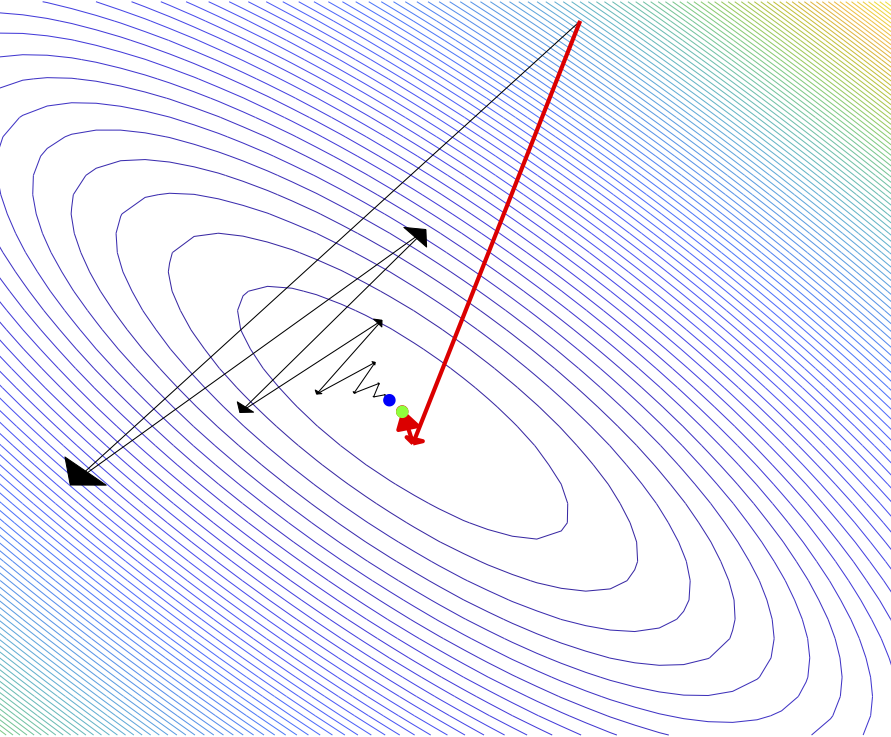M. Pilanci
Fast Randomized Algorithms for Convex Optimization and Statistical Estimation
PhD Thesis, University of California, Berkeley, 2016
machine learning convex optimization convex relaxations randomized algorithms
PDF

2015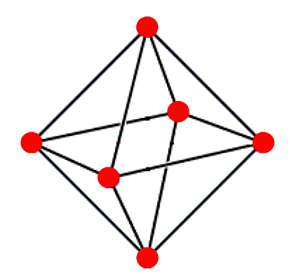M. Pilanci, M. J. Wainwright, and L. E. Ghaoui
Sparse learning via Boolean relaxations
Mathematical Programming, 151(1), 2015
sparse regression sparse classification randomized rounding
DOI PDF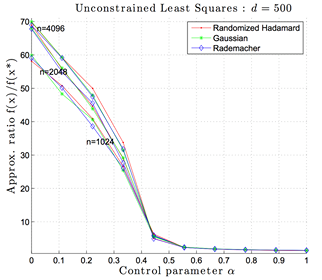M. Pilanci and M.J. Wainwright
Randomized Sketches of Convex Programs With Sharp Guarantees
IEEE Transactions on Information Theory, 61(9), 2015
random projection regression compressed sensing data privacy
DOI arXiv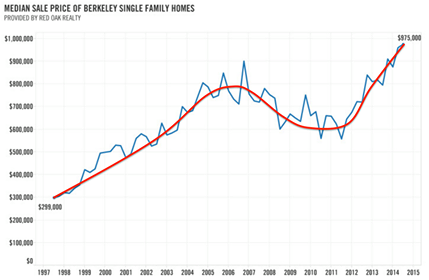Y. Yang, M. Pilanci, and M. J. Wainwright
Randomized sketches for kernels: Fast and optimal non-parametric regression
Annals of Statistics, Volume 45, Number 3 (2017), 991-1023.
kernel regression smoothing random projection Rademacher complexity
DOI PDF arXiv

2014M. Pilanci and M. J. Wainwright
Randomized sketches of convex programs with sharp guarantees
2014 IEEE International Symposium on Information Theory (ISIT), 2014
random projection convex optimization
DOI arXiv

2012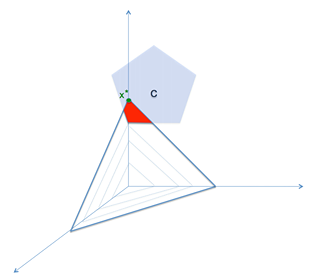M. Pilanci, L. E. Ghaoui, and V. Chandrasekaran
Recovery of sparse probability measures via convex programming
Advances in Neural Information Processing Systems (NIPS), 2012
probability measures convex relaxation data clustering
PDF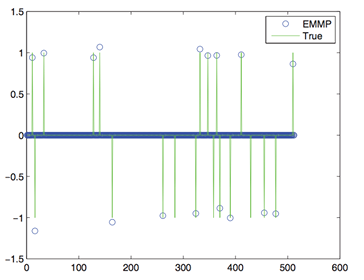A. C. Gurbuz, M. Pilanci, and O. Arikan
Expectation maximization based matching pursuit
Acoustics, Speech and Signal Processing (ICASSP), 2012 IEEE International Conference on, 2012
sparse approximation compressed sensing
DOI PDF

2011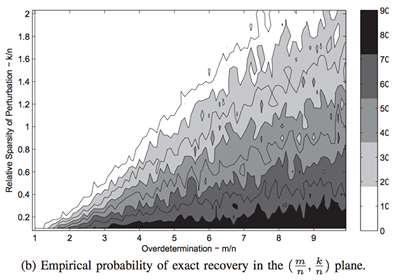M. Pilanci and O. Arikan
Recovery of sparse perturbations in least squares problems
Acoustics, Speech and Signal Processing (ICASSP), 2011 IEEE International Conference on,2011
robust optimization regression sparsity
DOI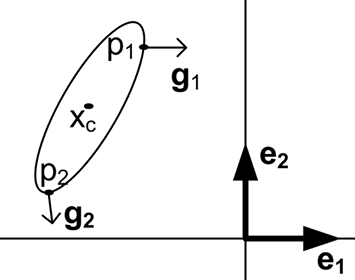A. C. Gurbuz, M. Pilanci, and O. Arikan
Sparse signal reconstruction with ellipsoid enlargement
Signal Processing and Communications Applications (SIU), 2011 IEEE 19th Conference on, 2011
sparsity compressed sensing signal processing
DOI

2010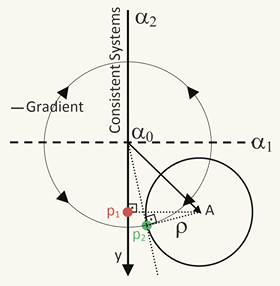M. Pilanci, O. Arikan, and M. C. Pinar
Structured least squares problems and robust estimators
IEEE Transactions on Signal Processing, 58(5), 2010
robust optimization robust estimation
DOI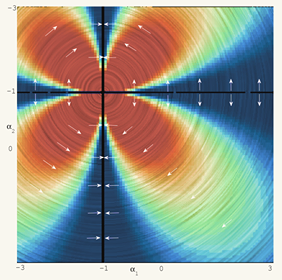M. Pilanci
Uncertain linear equations
MS Thesis, Bilkent University, 2010
robust optimization compressed sensing coding and information theory polar codes
PDF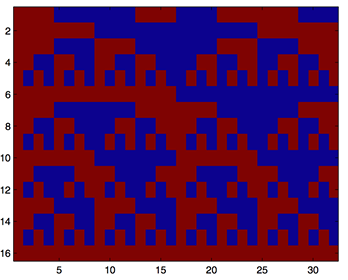M. Pilanci, O. Arikan, and E. Arikan
Polar compressive sampling: A novel technique using Polar codes
Signal Processing and Communications Applications Conference (SIU), 2010 IEEE 18th,2010
compressed sensing coding and information theory polar codes signal processing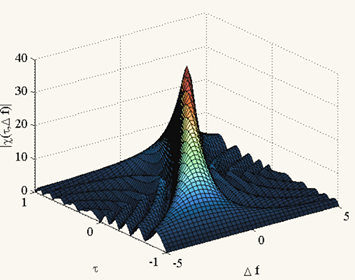B. Guldogan, M. Pilanci, and O. Arikan
Compressed sensing on ambiguity function domain for high resolution detection
Signal Processing and Communications Applications Conference (SIU), 2010 IEEE 18th, 2010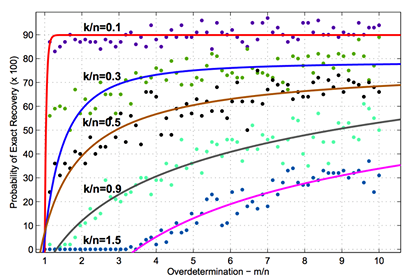M. Pilanci and O. Arikan
Compressive sampling and adaptive multipath estimation
Signal Processing and Communications Applications Conference (SIU), 2010 IEEE 18th, 2010
wireless communications compressed sensing

2009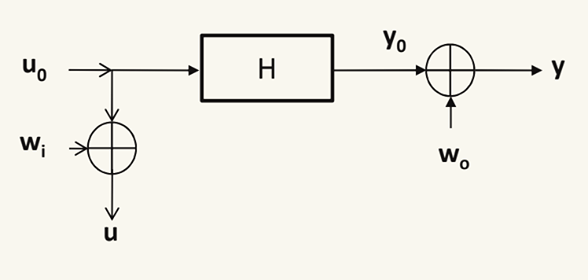M. Pilanci, O. Arikan, B. Oguz, and M. Pinar
A novel technique for a linear system of equations applied to channel equalization
Signal Processing and Communications Applications Conference, 2009. SIU 2009. IEEE 17th, 2009
wireless communications robust optimization
DOI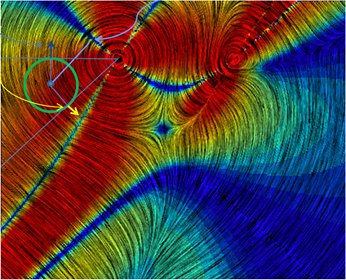M. Pilanci, O. Arikan, B. Oguz, and M. Pinar
Structured least squares with bounded data uncertainties
Acoustics, Speech and Signal Processing, 2009. ICASSP 2009. IEEE International Conference on, 2009
robust optimization regression statistical estimation
DOI